NCERT Exemplar Class 8 Maths Solutions for Chapter 6 - Visualising Solid Shapes

NCERT Exemplar Class 8 Maths Solutions for Chapter 6 - PDF Download Free Download

NCERT Exemplar Class 8 Maths Chapter 6 Visualising Solid Shapes, is provided here for students to prepare for exams. These exemplars solutions are designed by experts in accordance with CBSE syllabus(2018-2019), which covers all the topics of Maths chapter 6 of 8th standard.

The chapter visualizing solid shapes is about learning different types of shapes in 2 and 3 dimensions. To master the concepts of this chapter students are advised to solve the questions from the NCERT exemplar problems for class 8 maths visualizing solid shapes.

Class 8 NCERT Exemplar For Visualising Solid Shapes

Solve exemplar problems for class 8, as it will help you to understand the concepts present in each chapter of Maths as well as Science subjects. Below is the list of topics covered in chapter 6 of the 8th class.

• Difference between 2D and 3D figures
• Different types of 3D shapes
• Faces, Edges and Vertices

Apart from these exemplars, we provide notes, exemplar books, NCERT Maths solutions for 8th standard and question papers to help students practice well for final exams. Students are advised to solve sample papers and previous year question papers which gives an idea of types of questions asked in the board exam from Visualising Solid Shapes.

Visit the below download link to get free PDF of NCERT exemplar for class 8 maths chapter 6.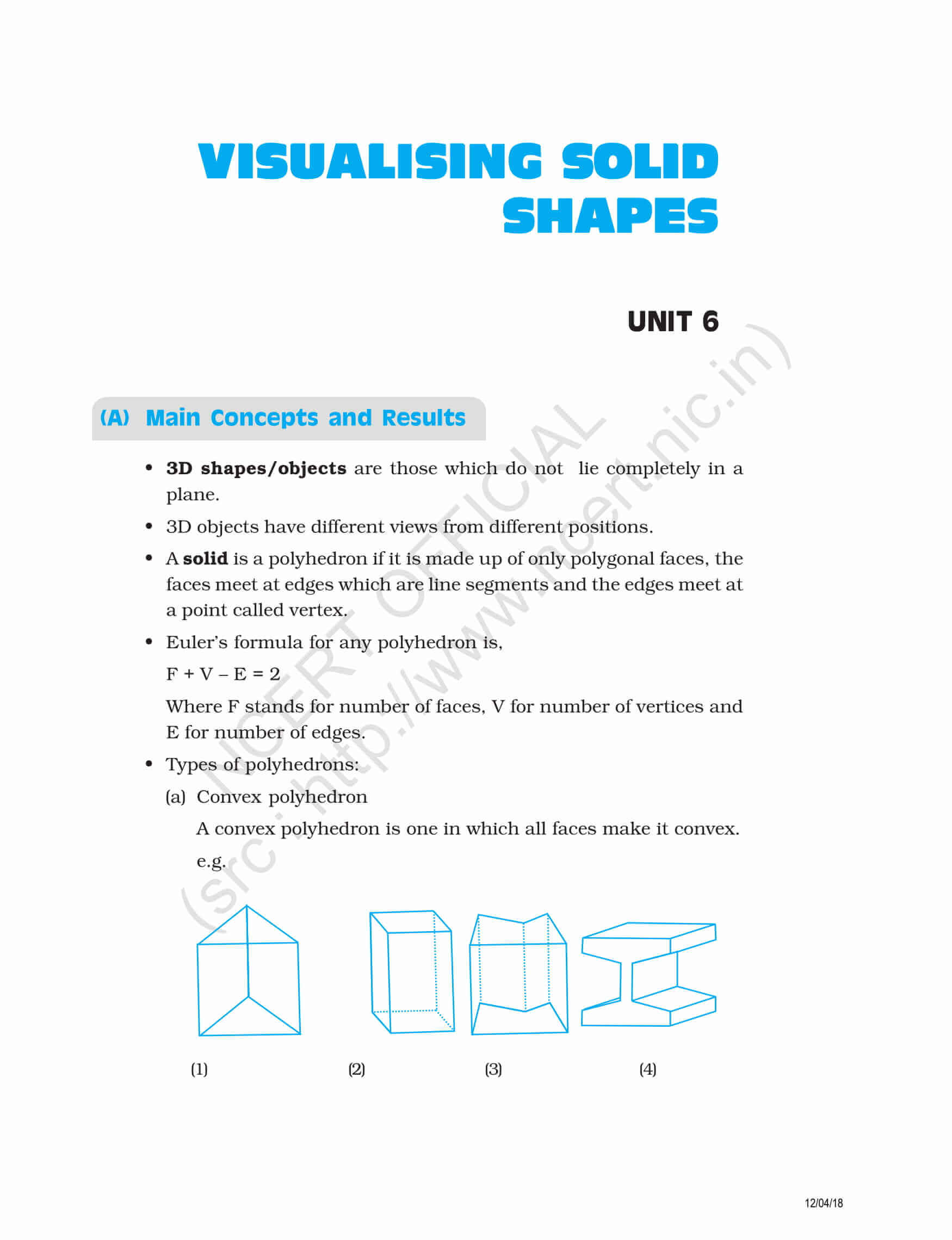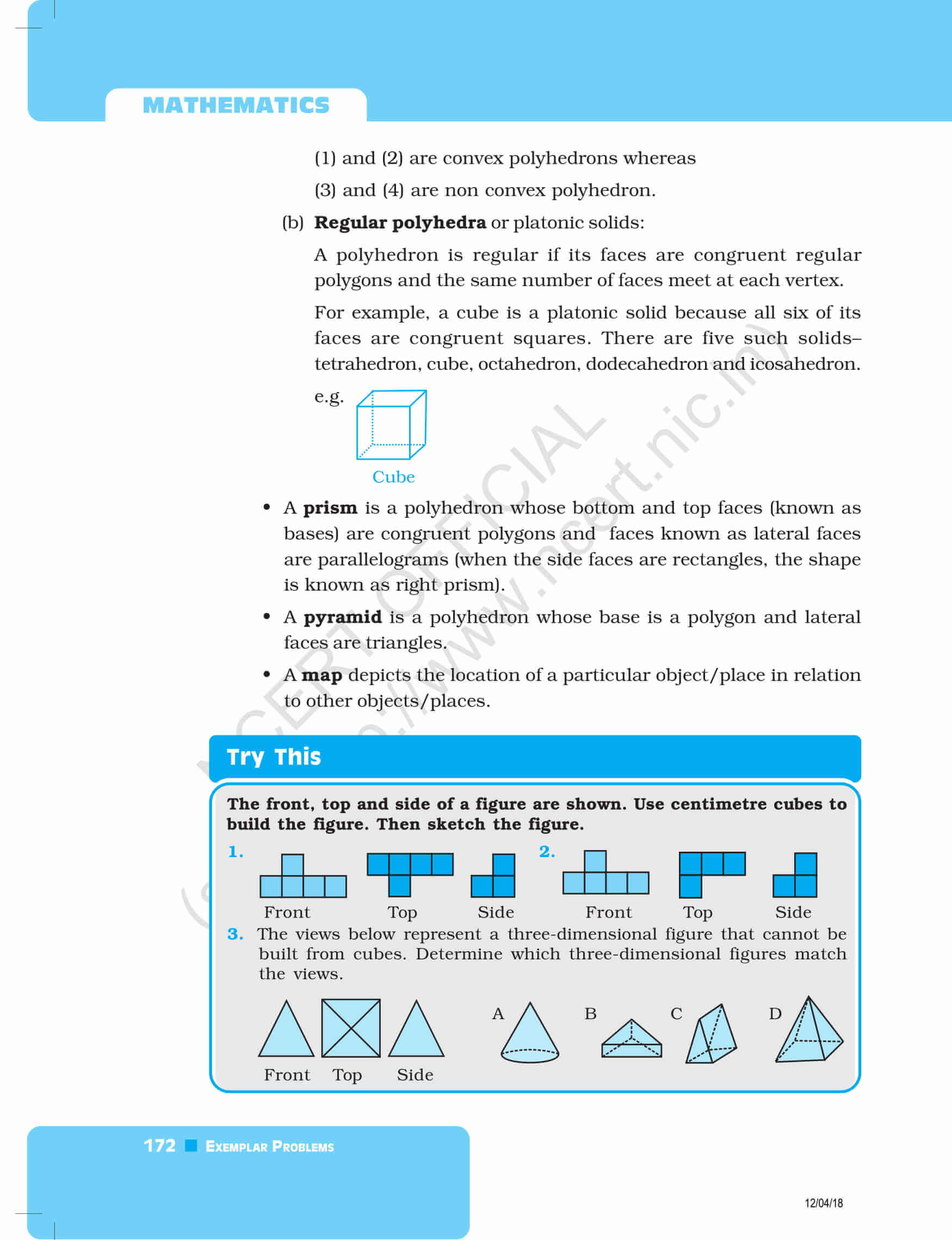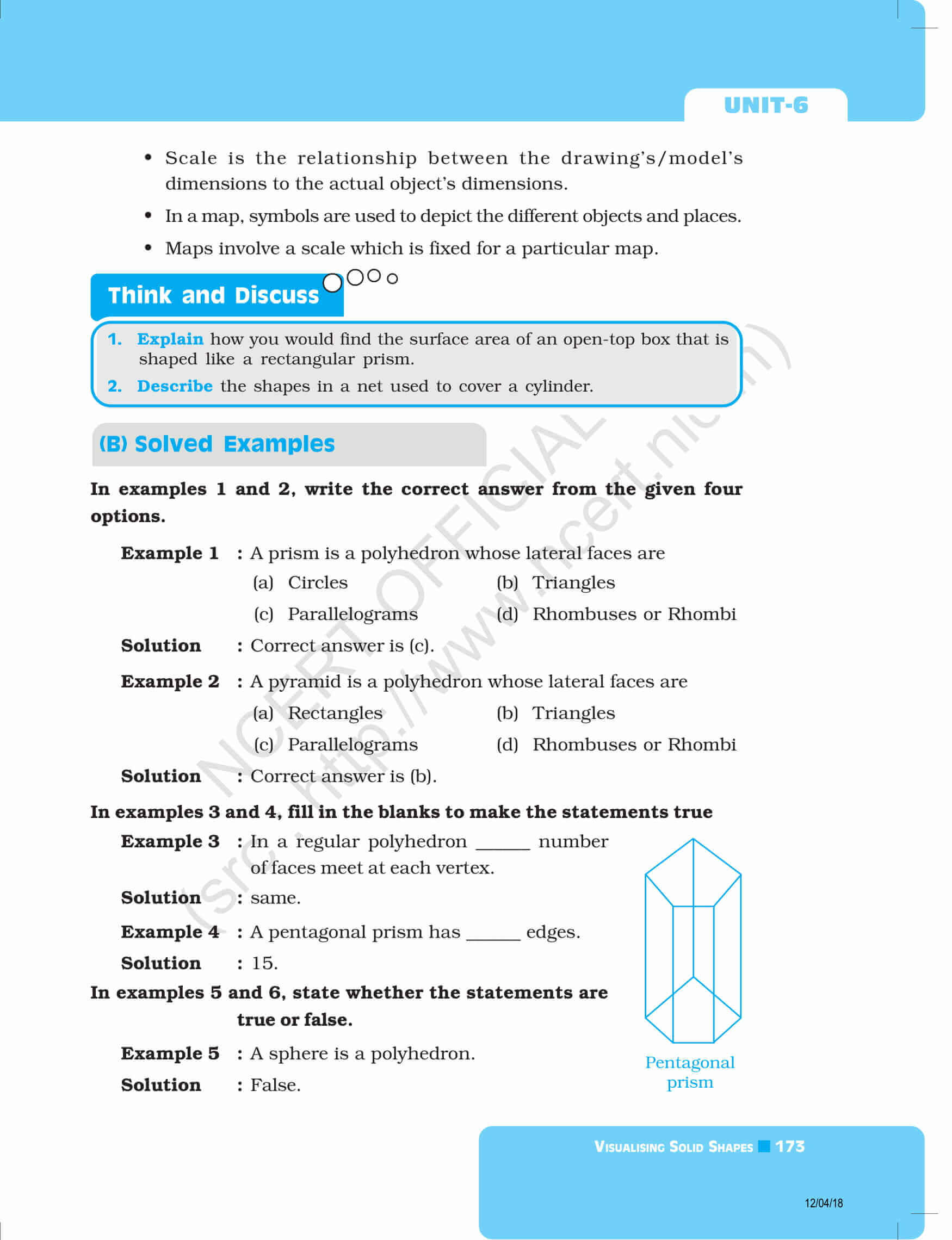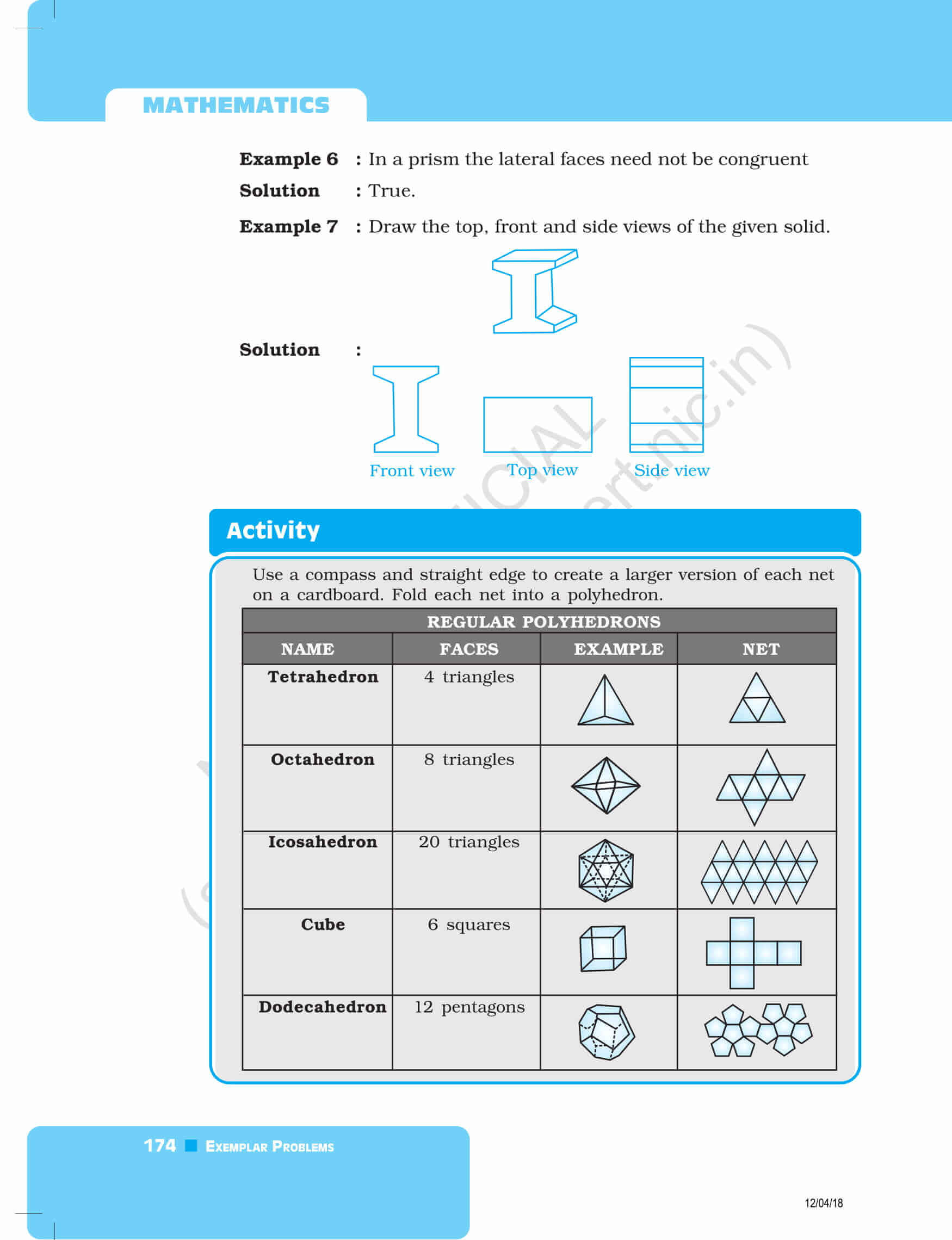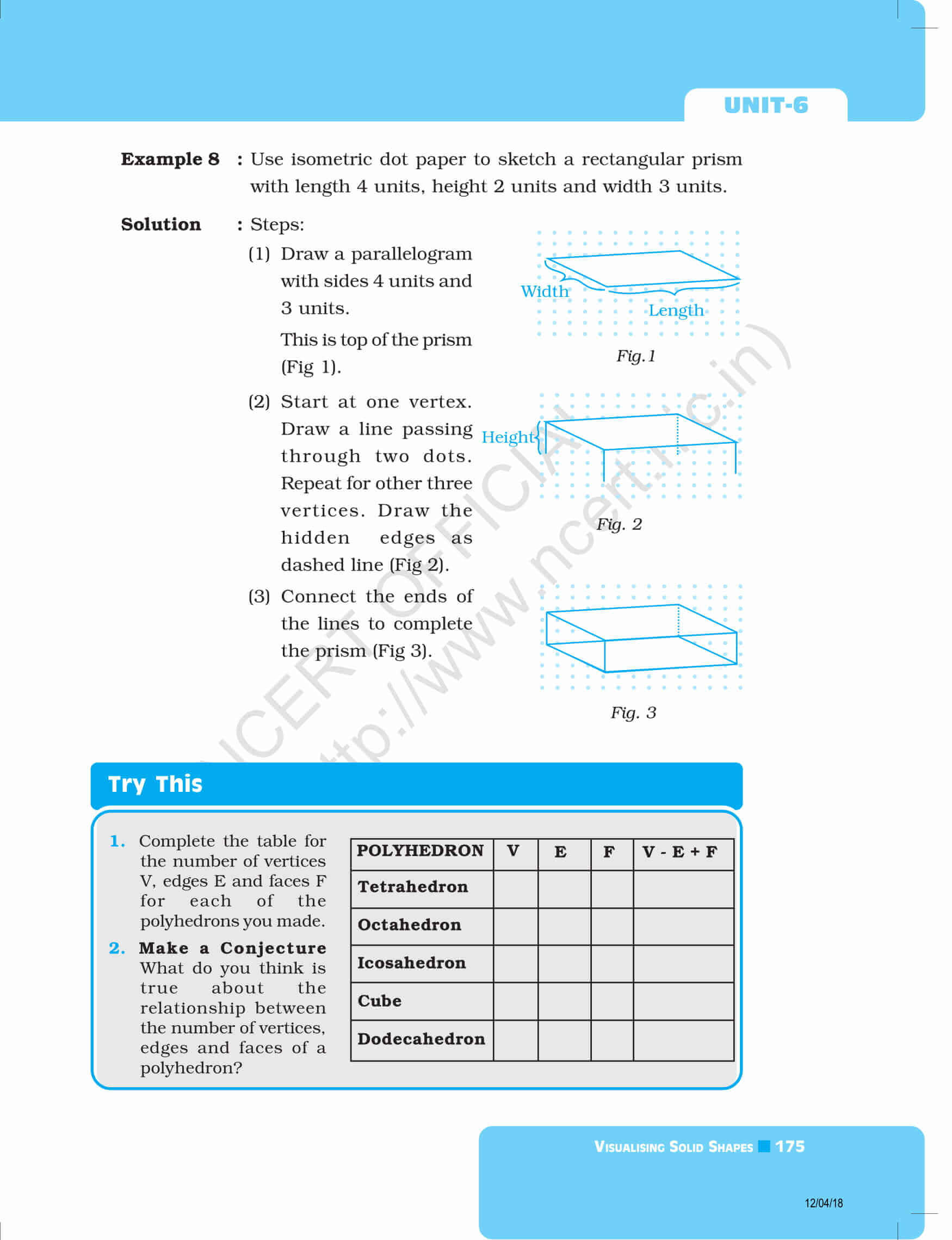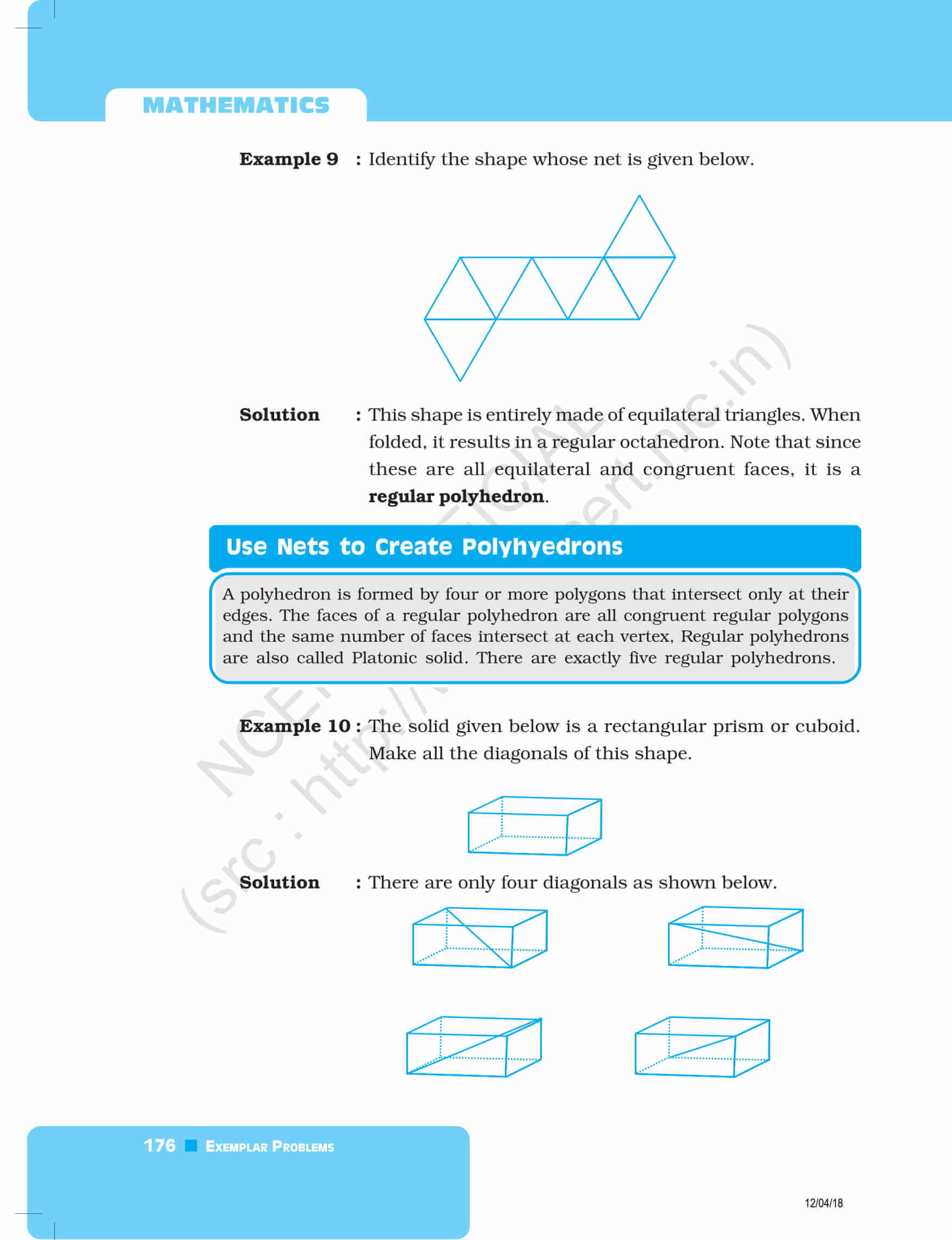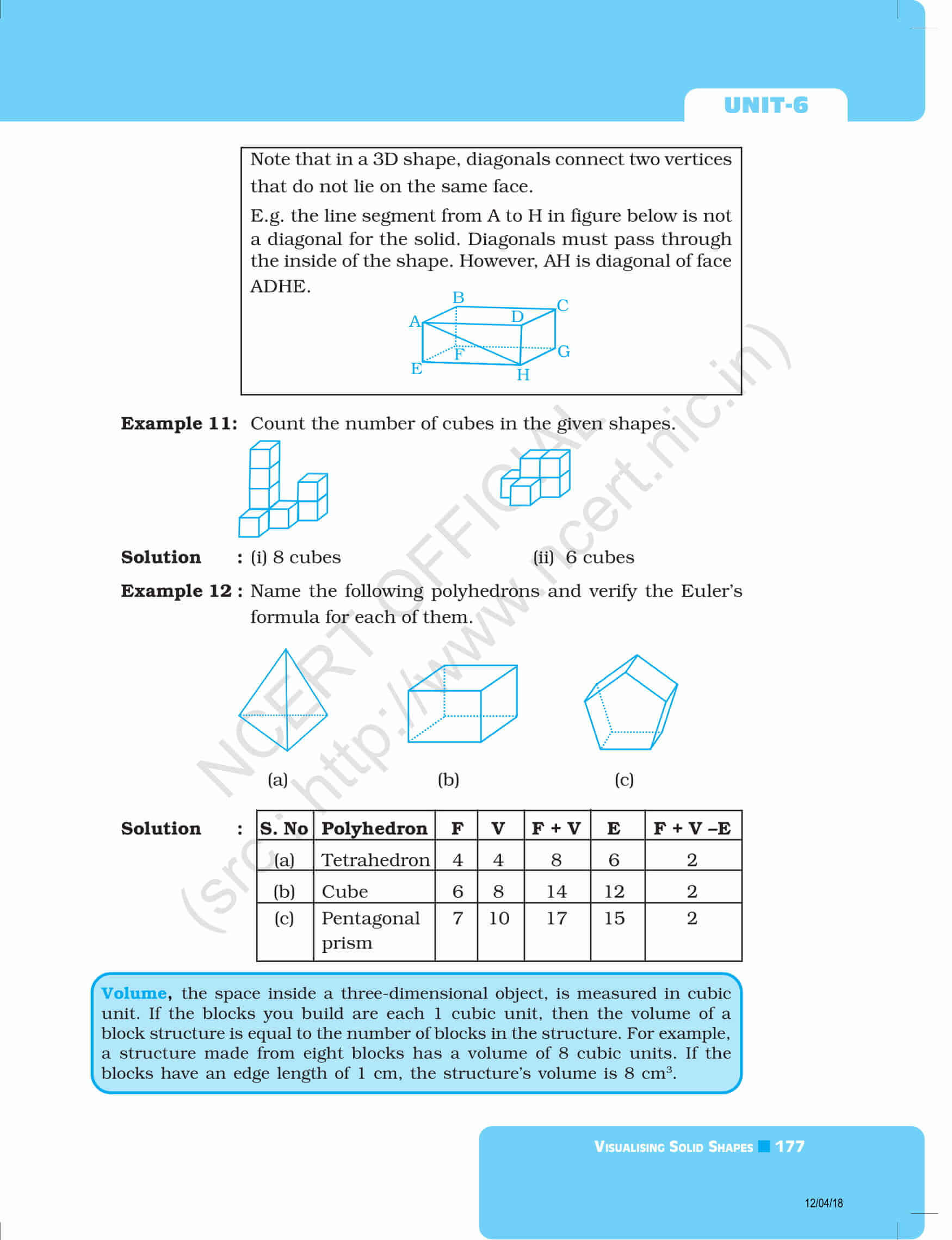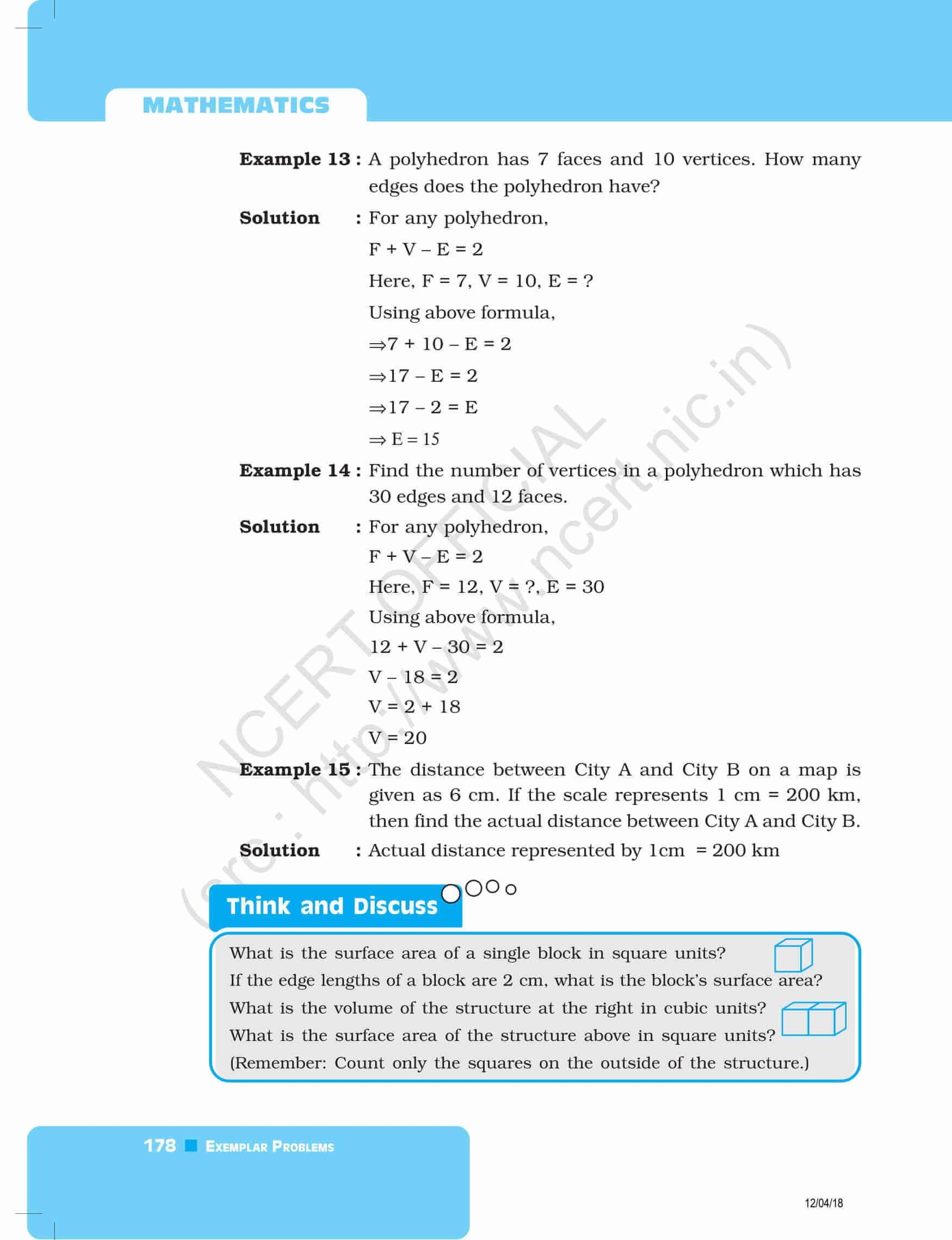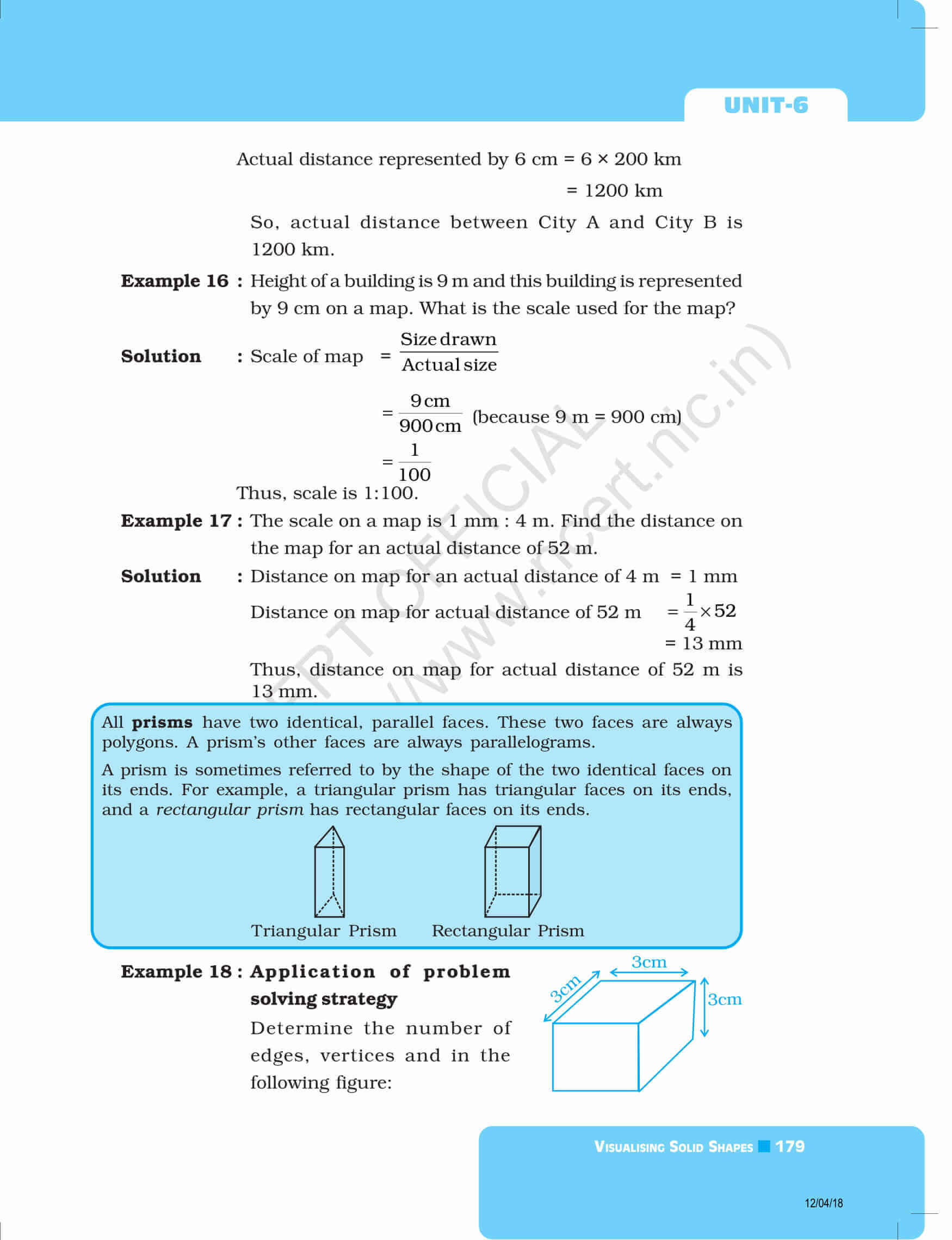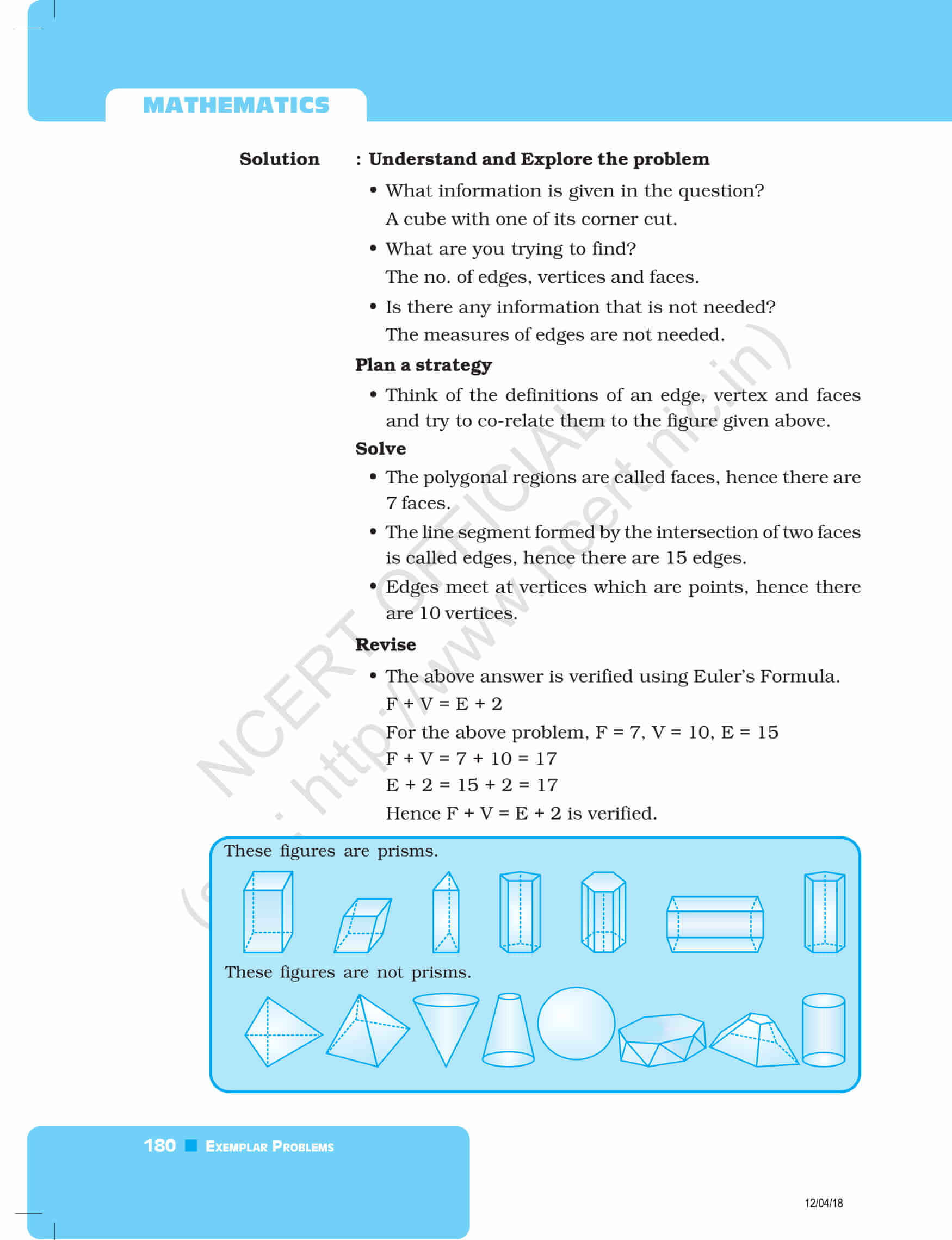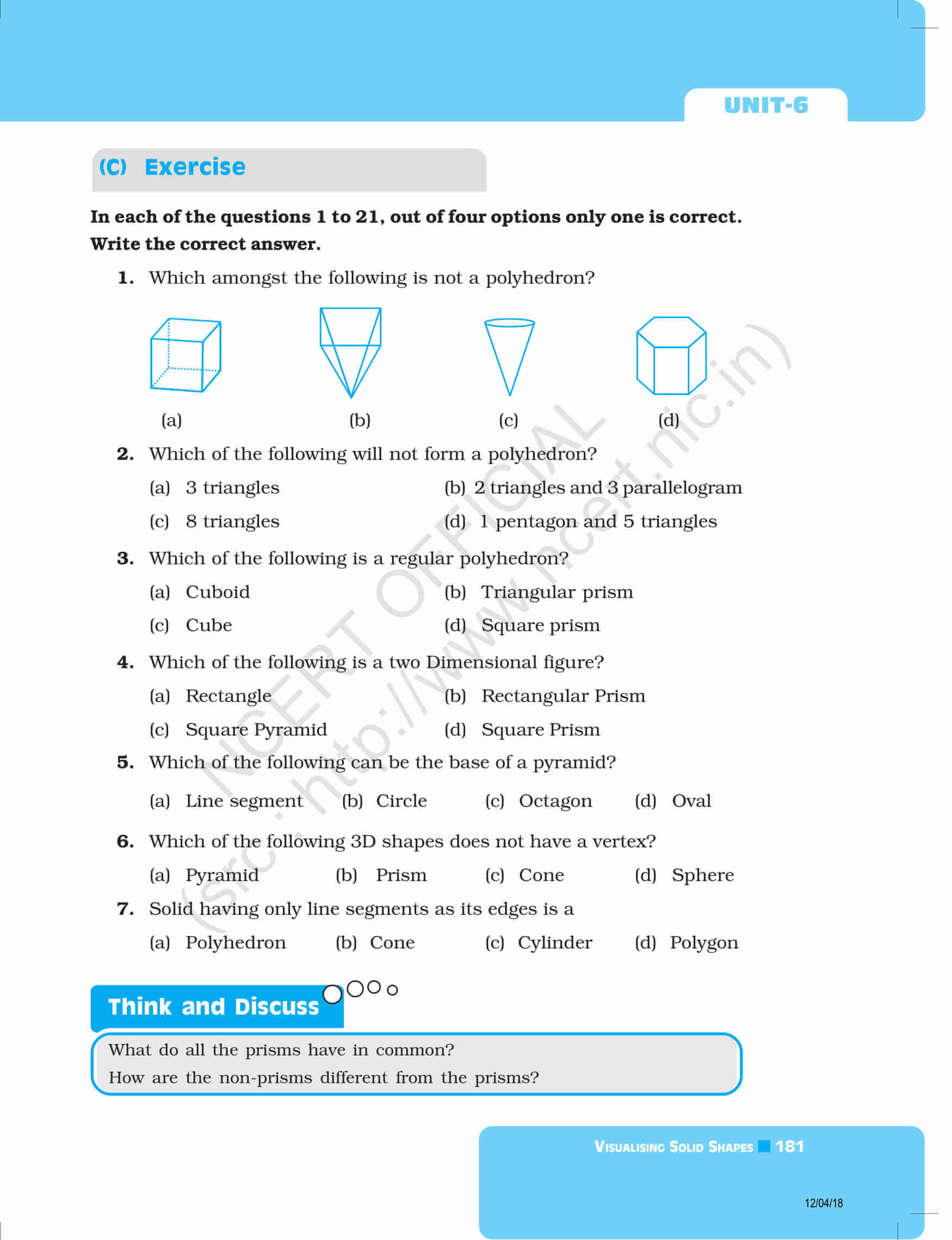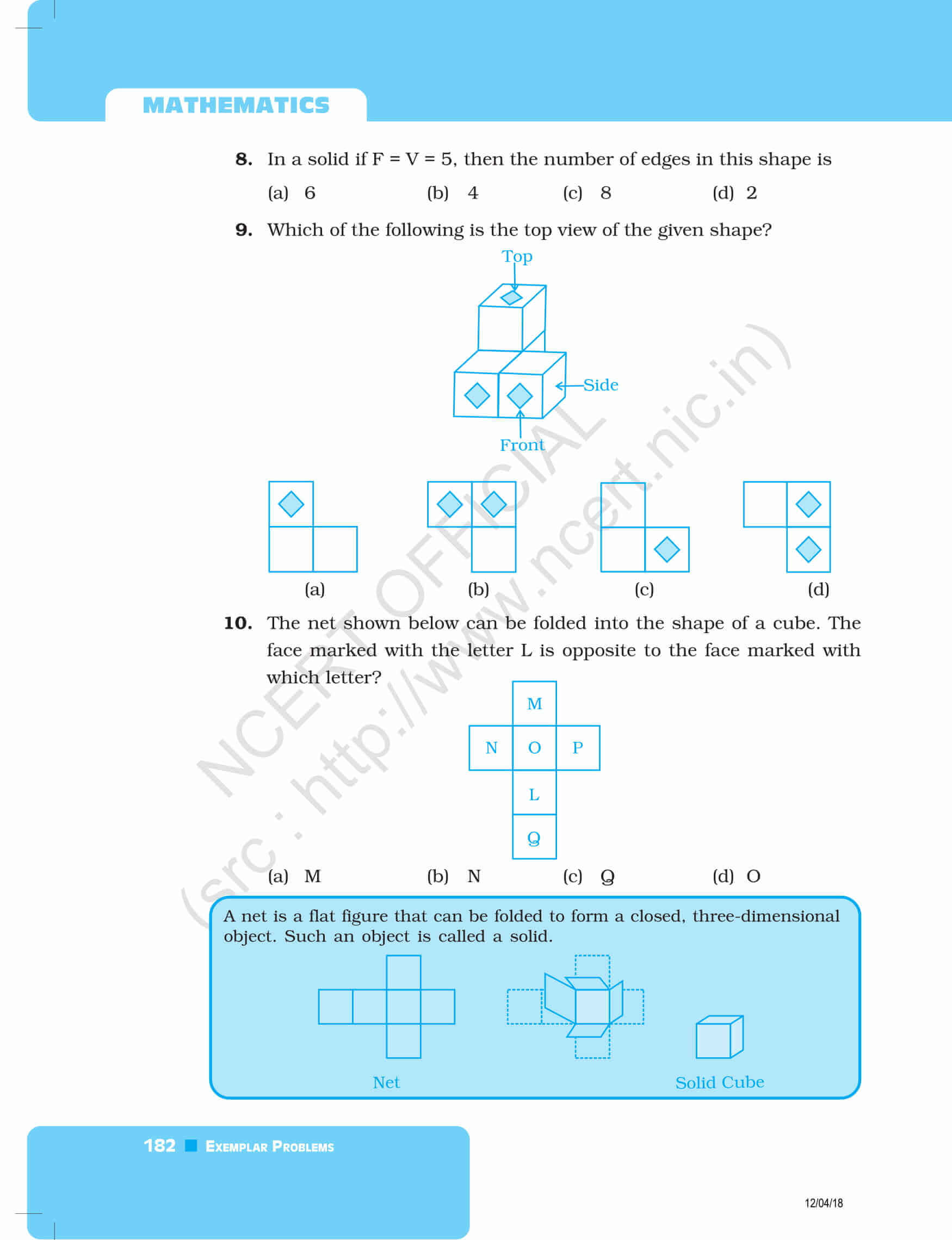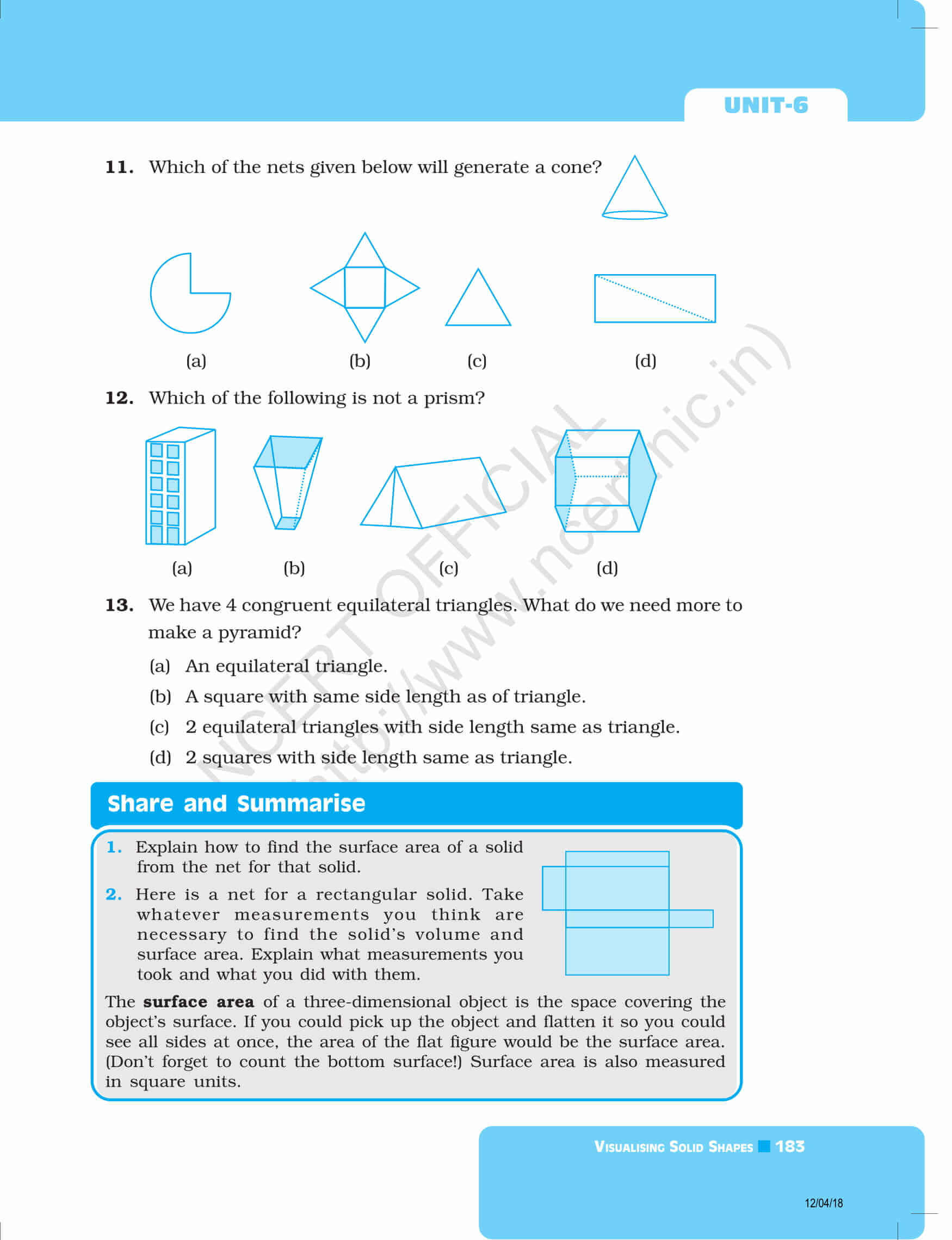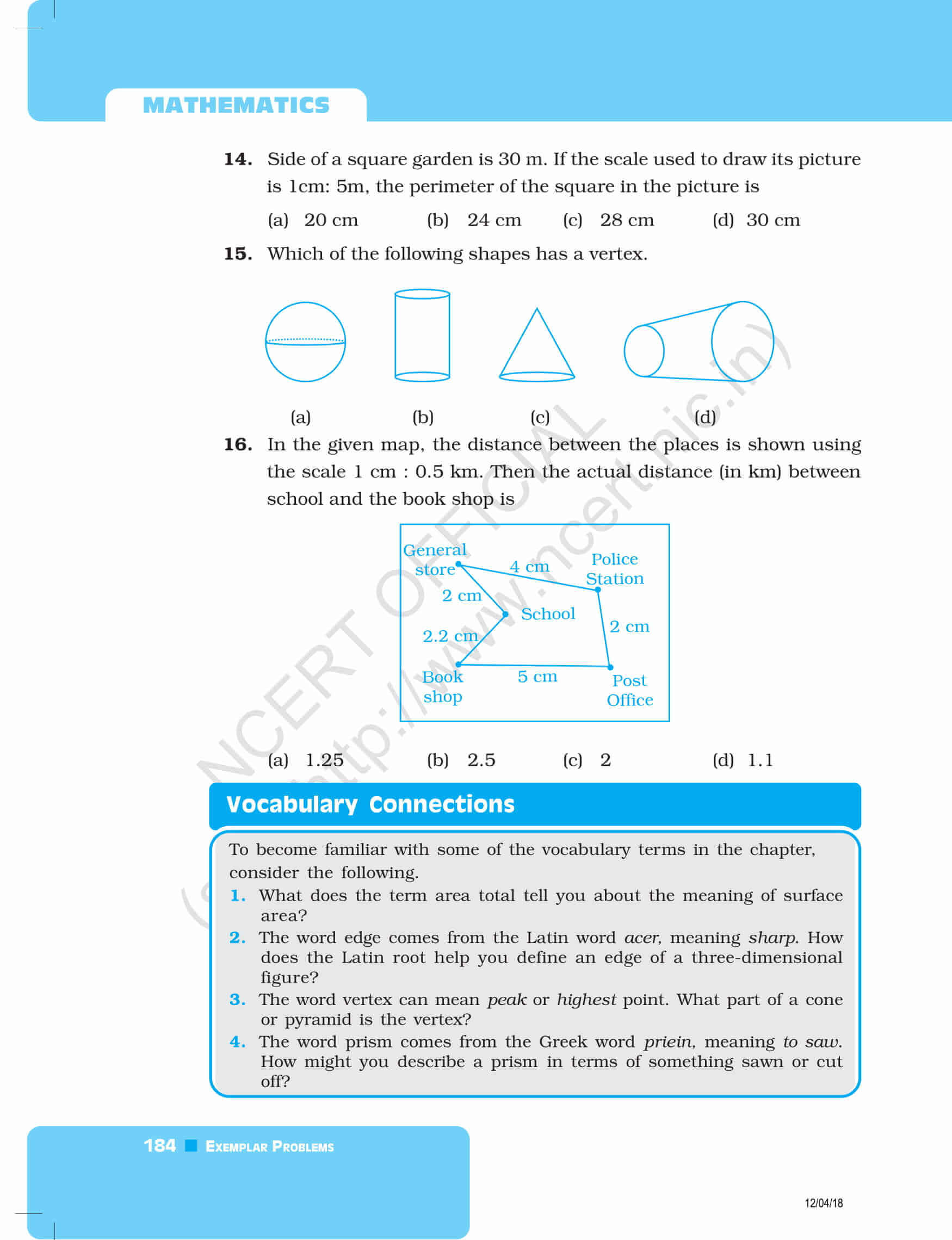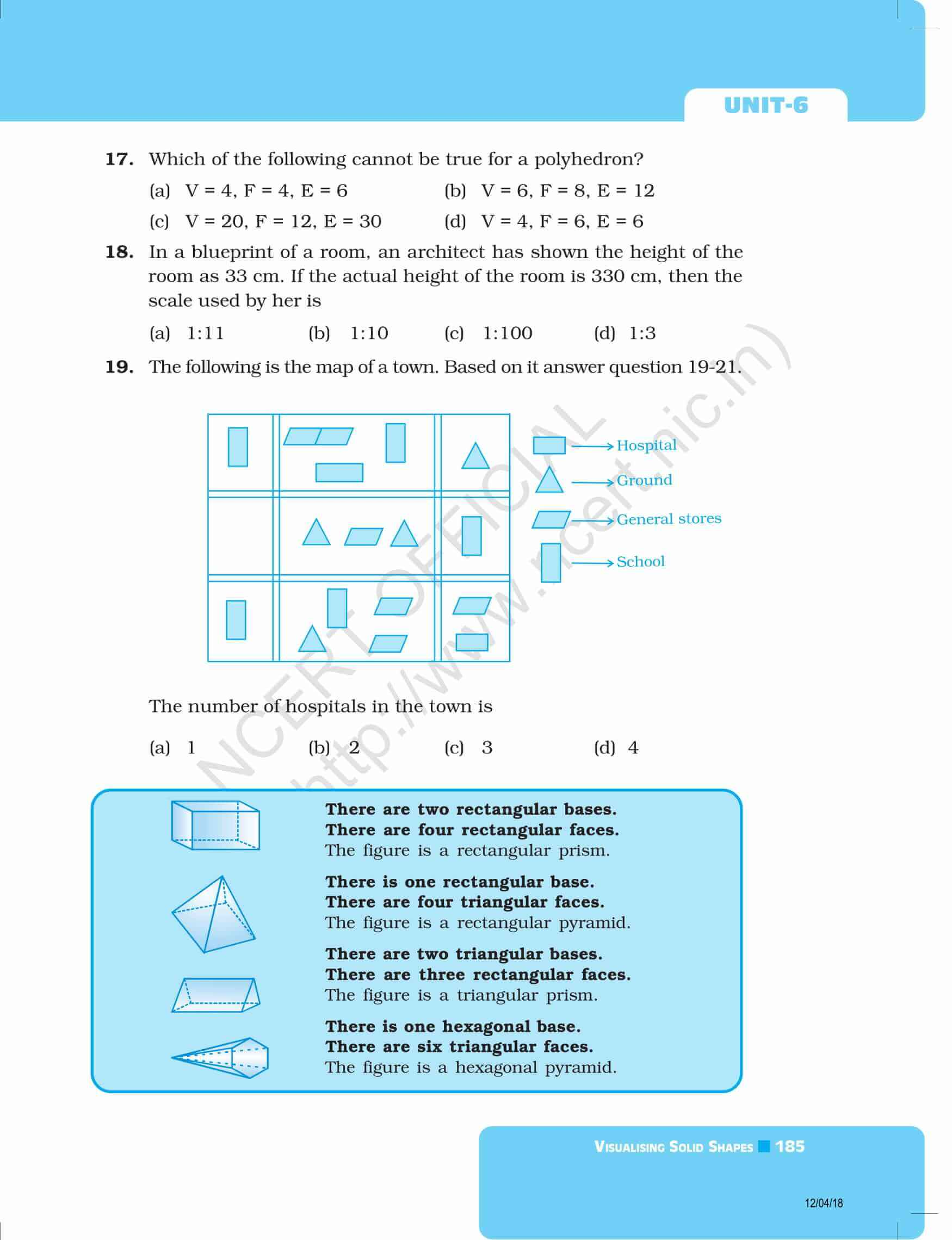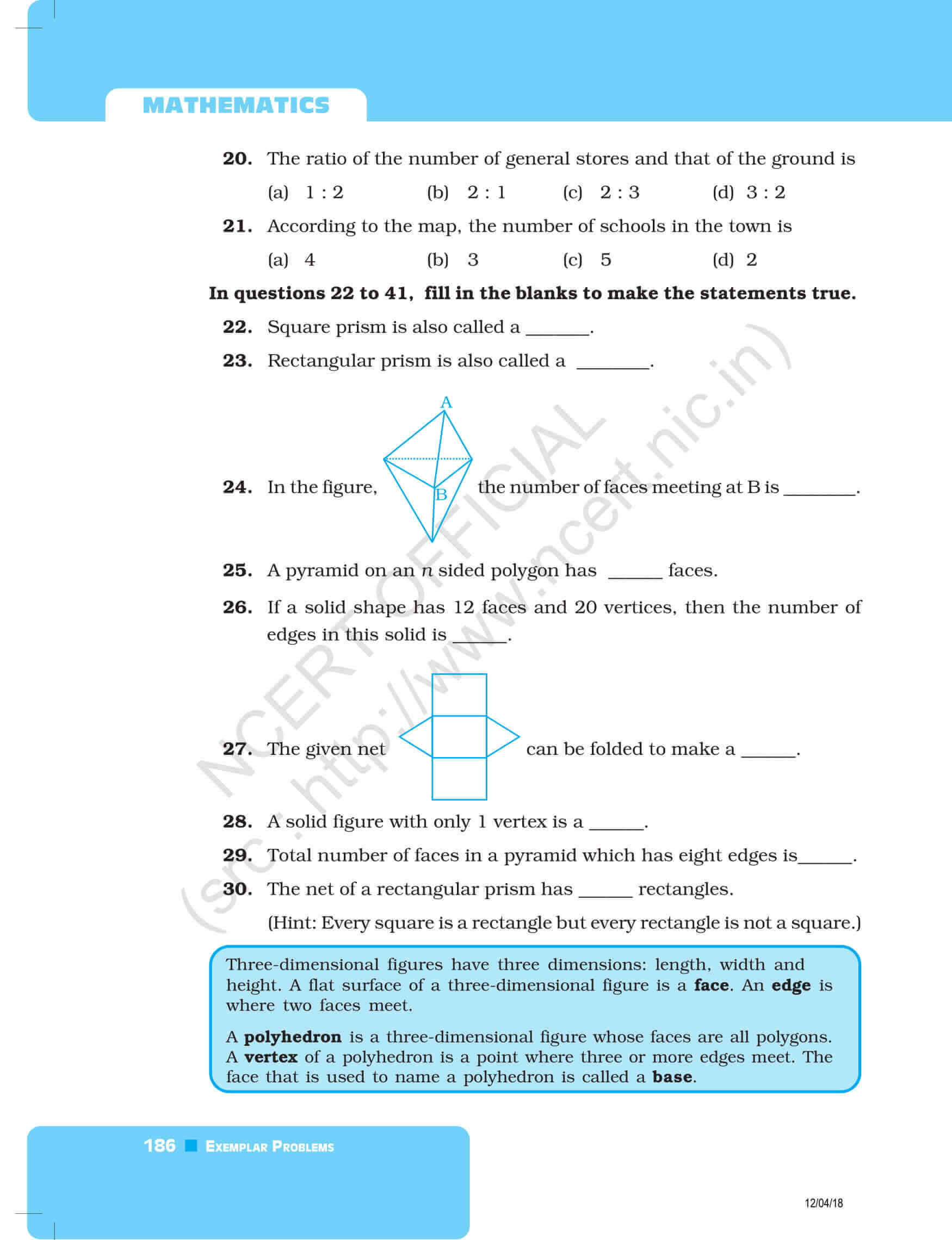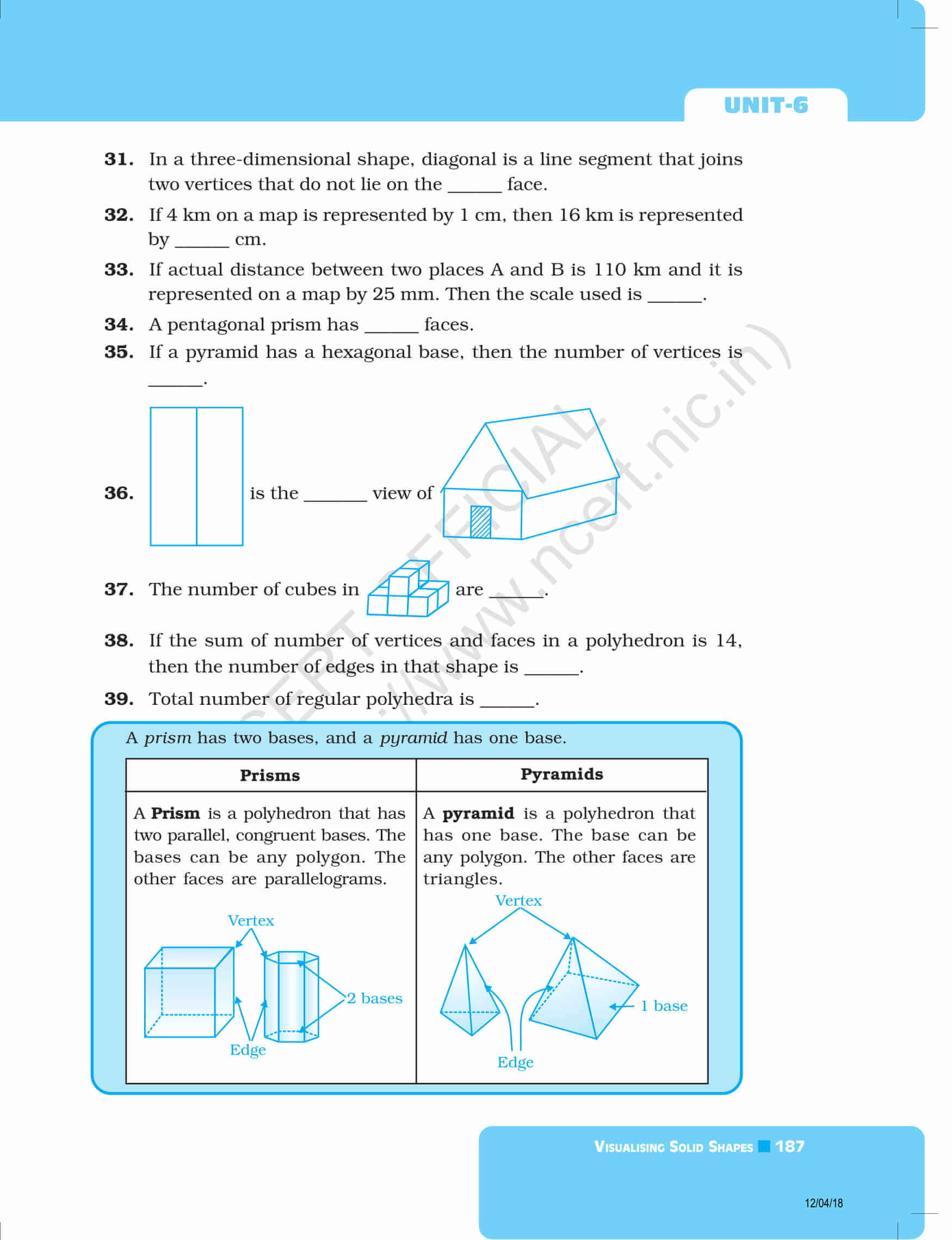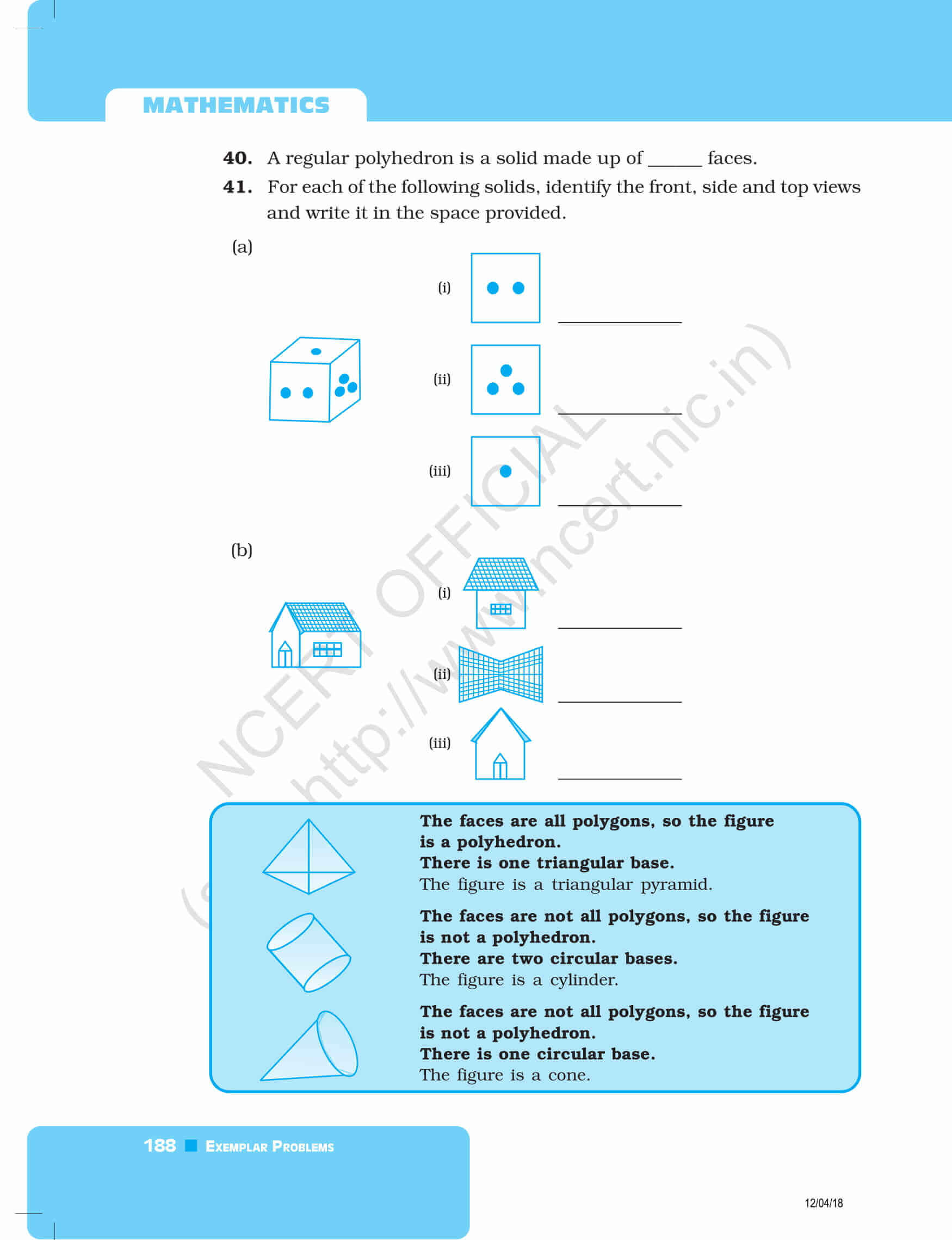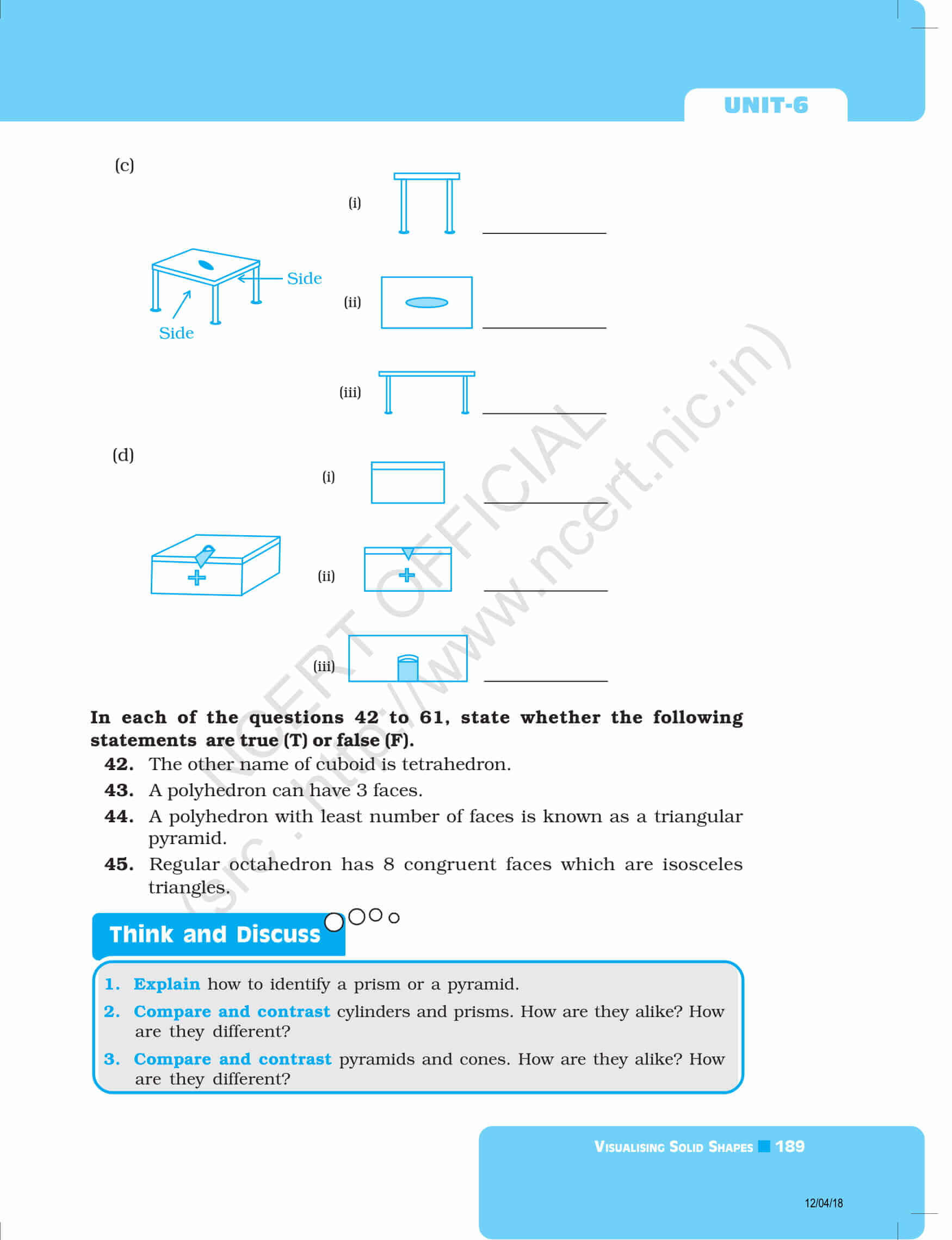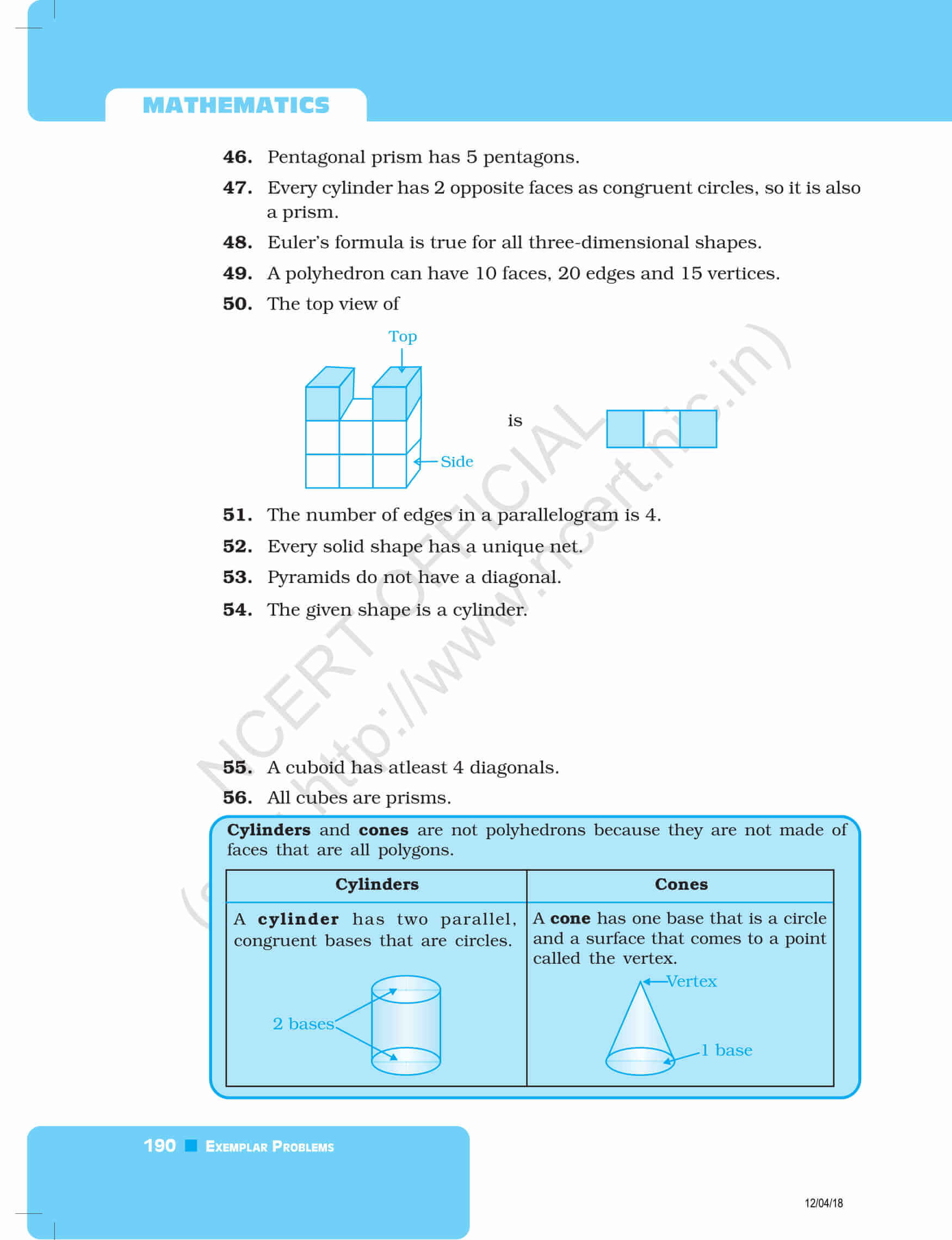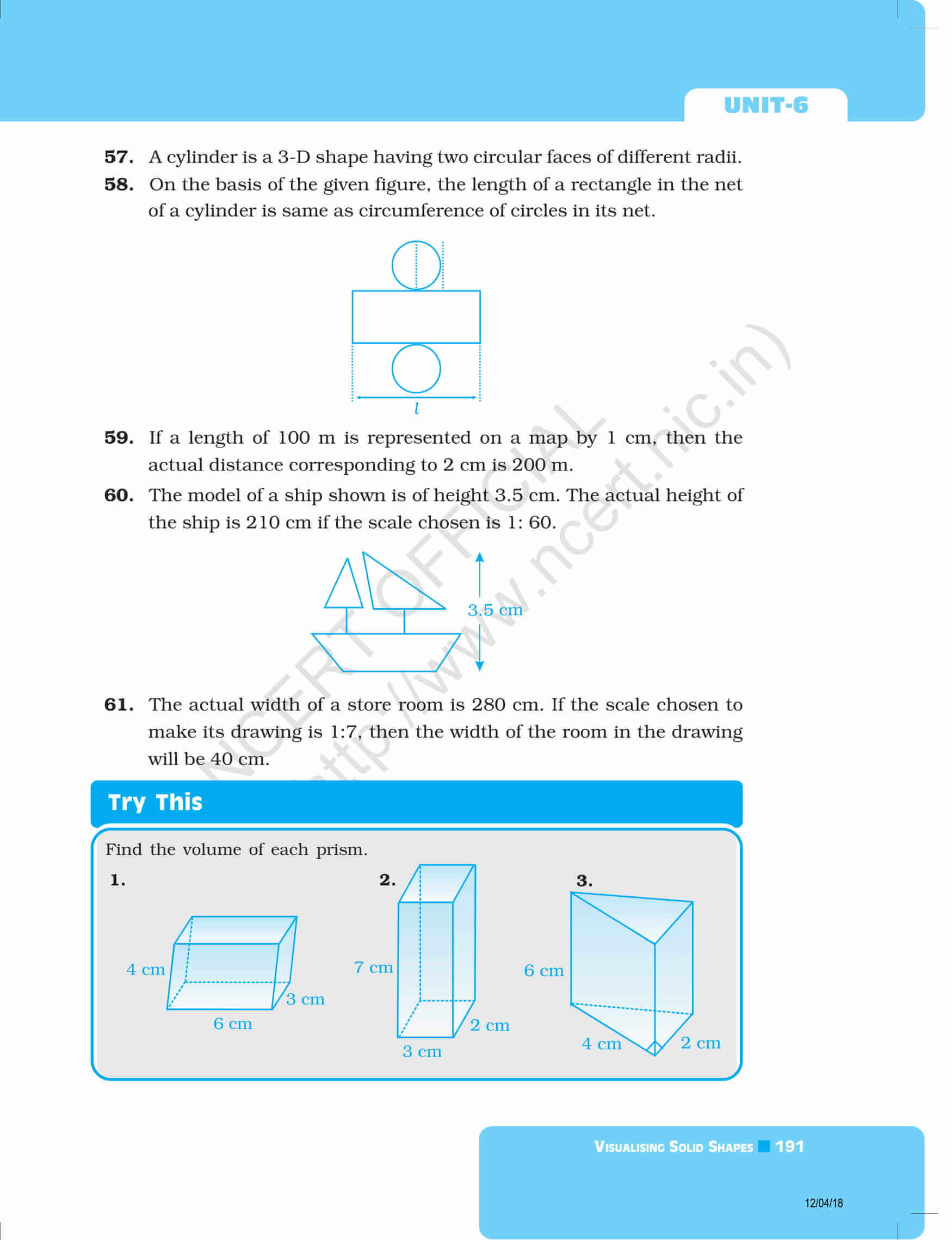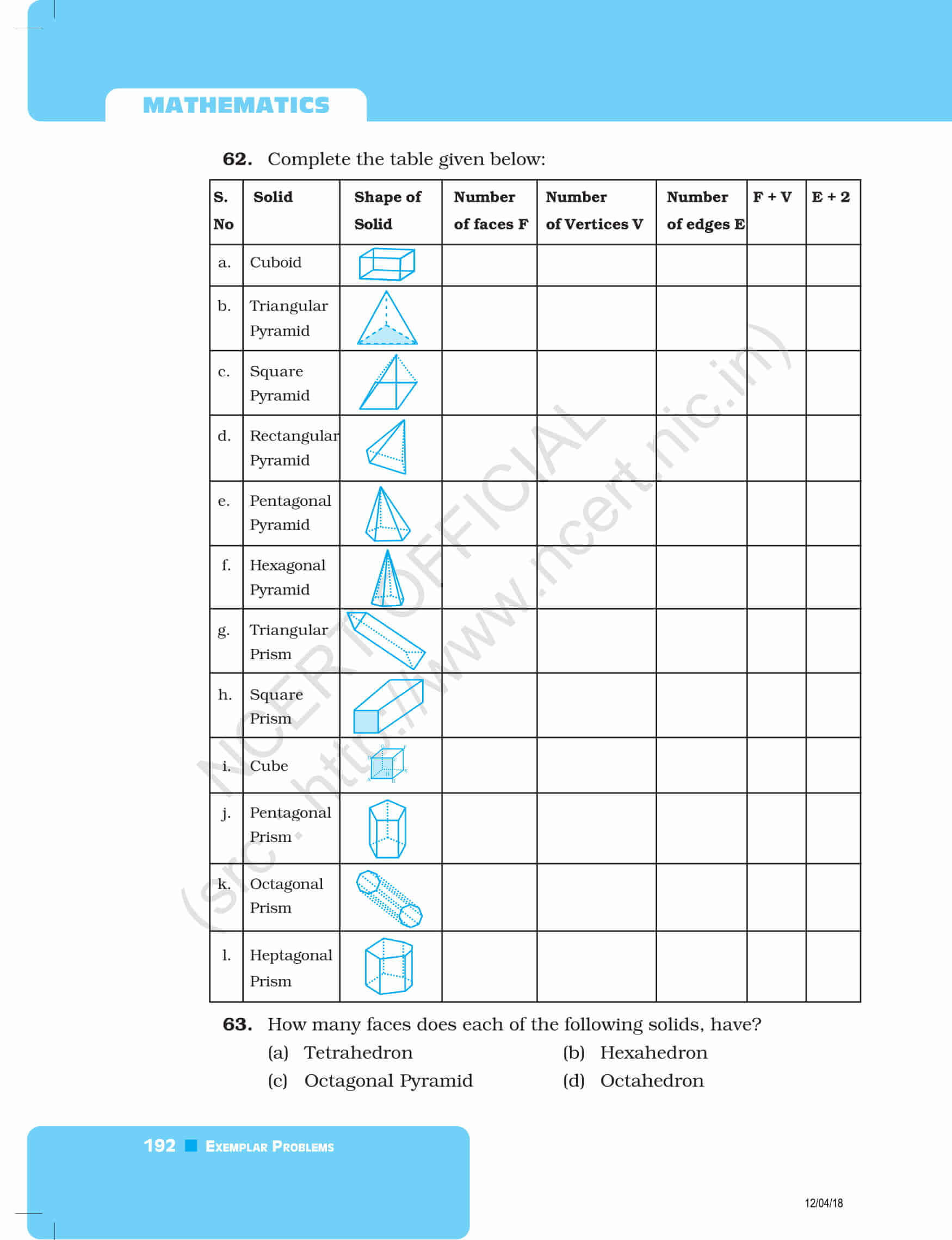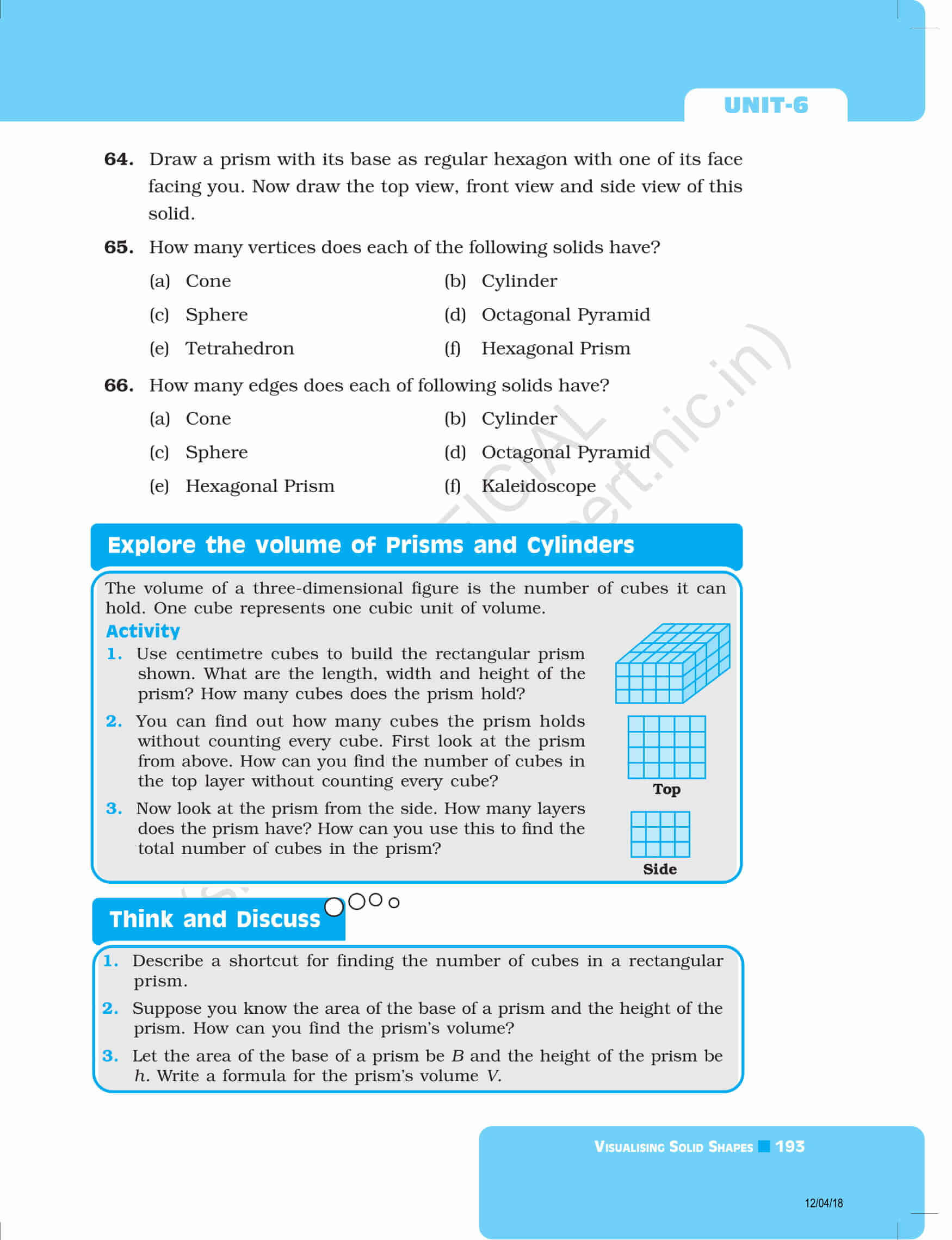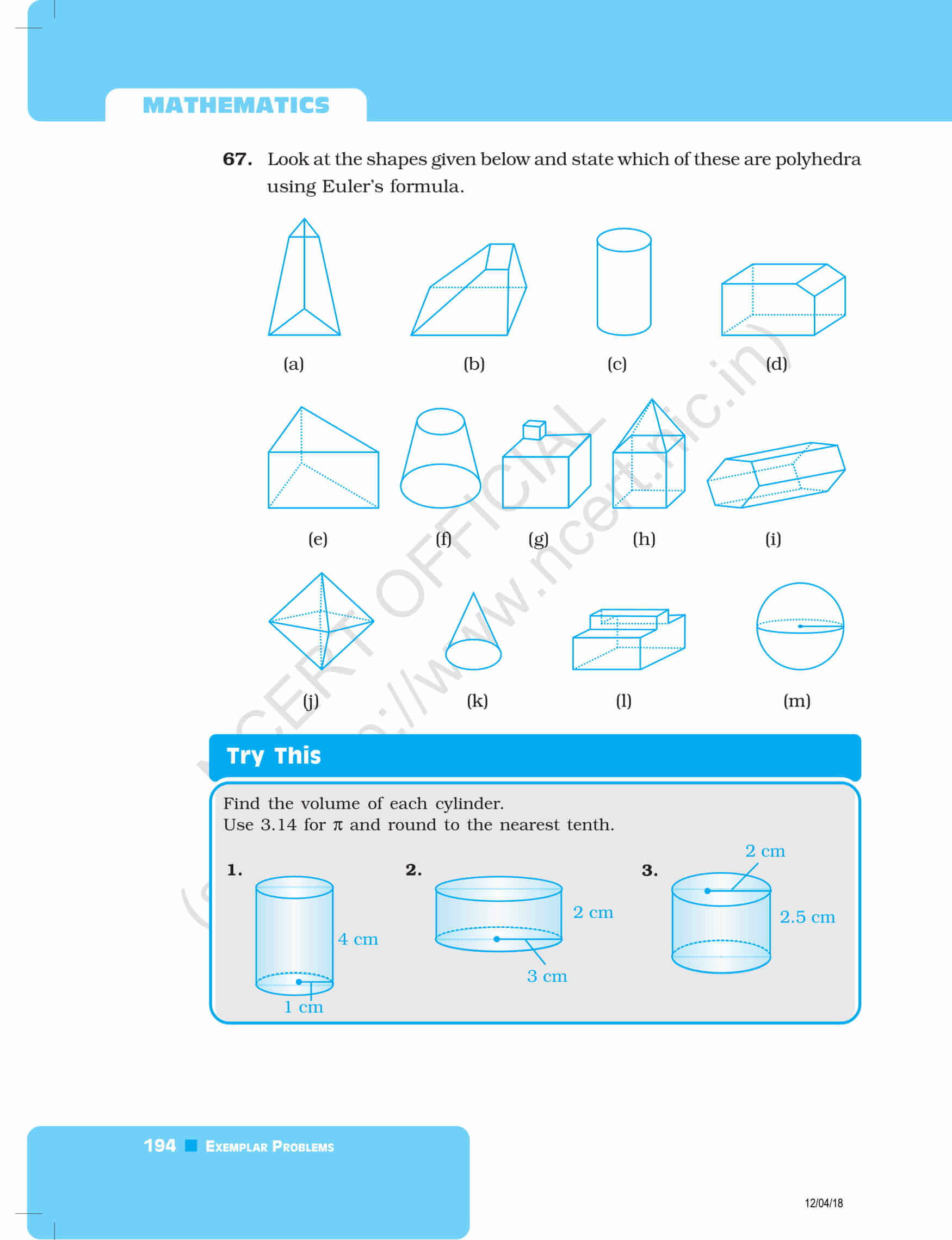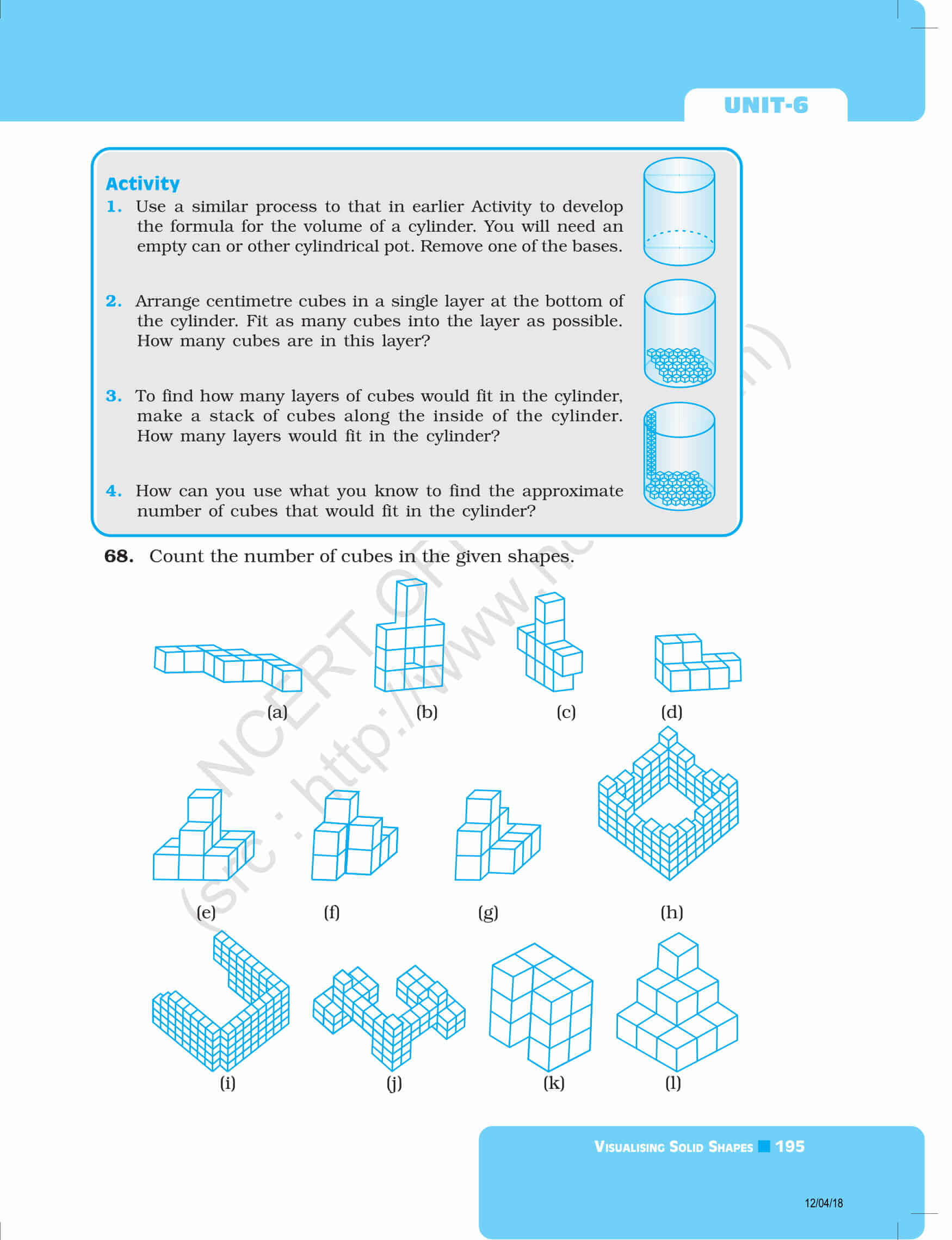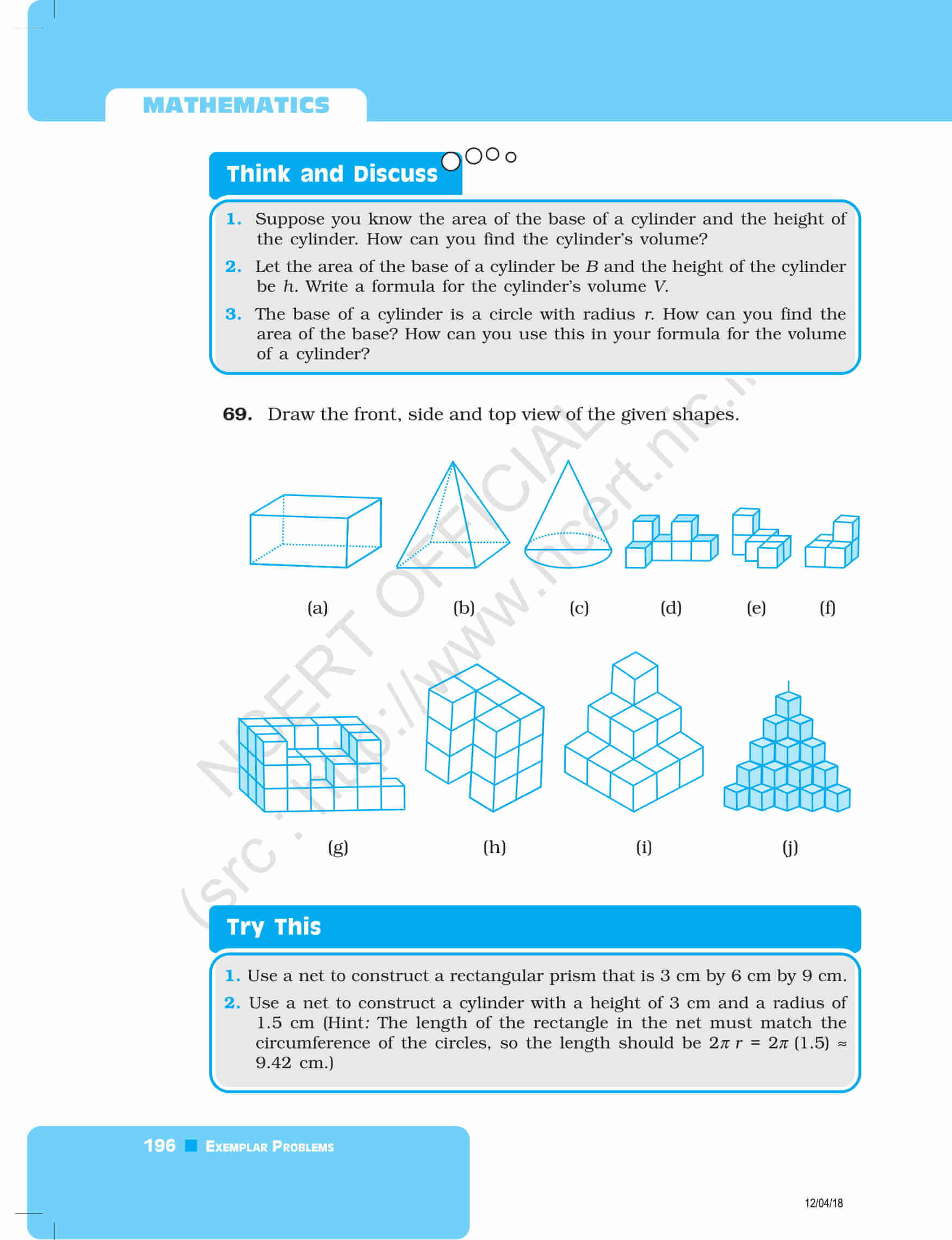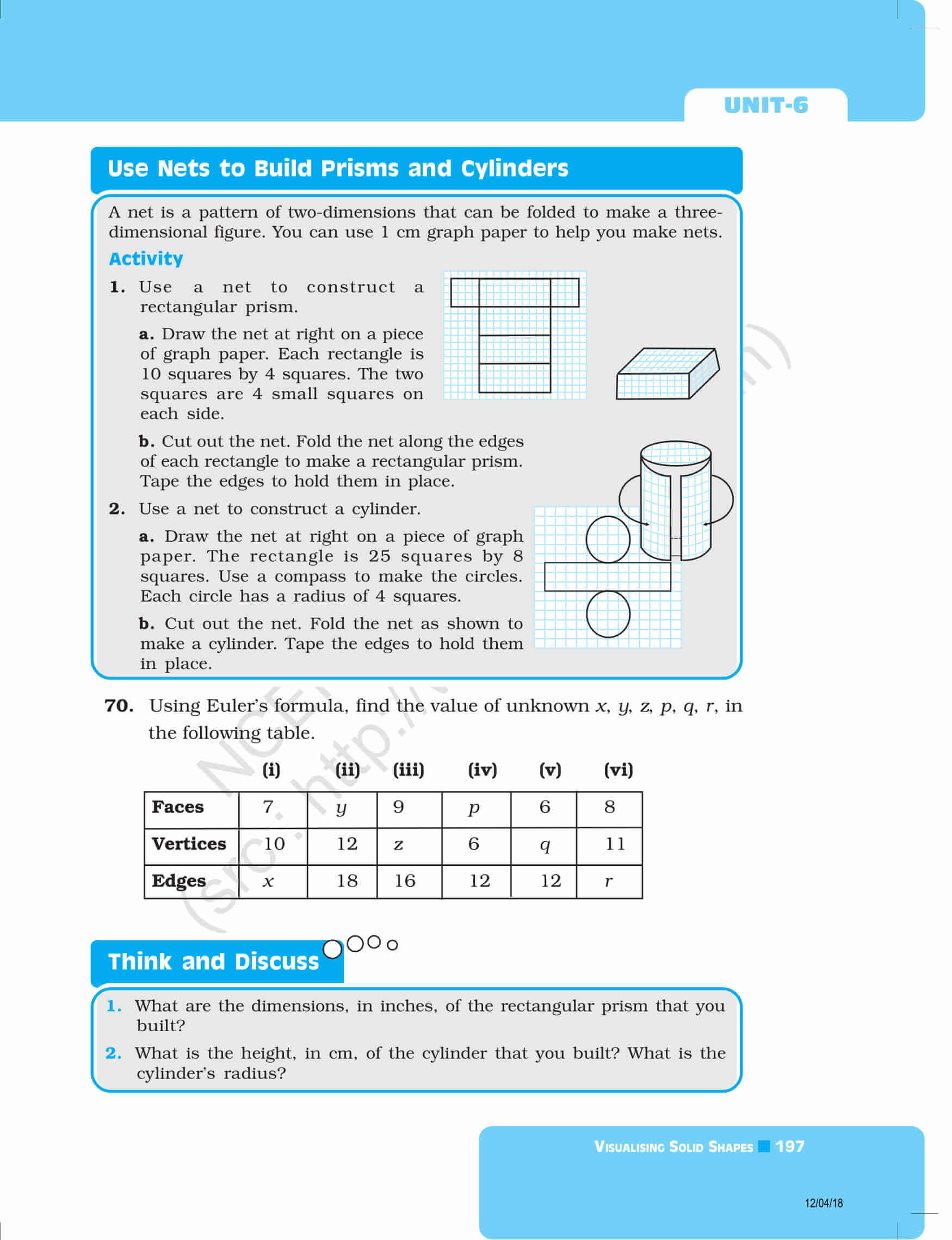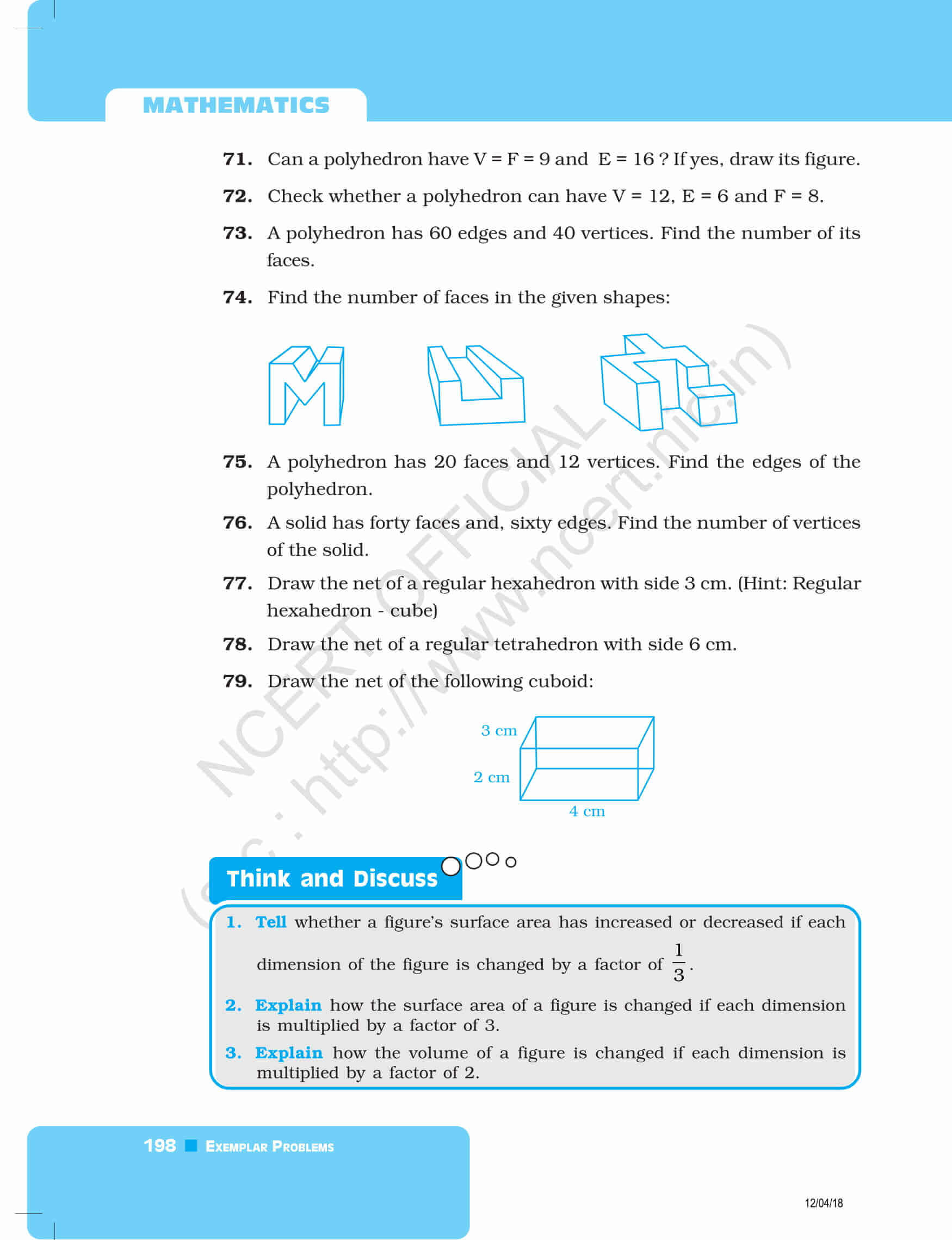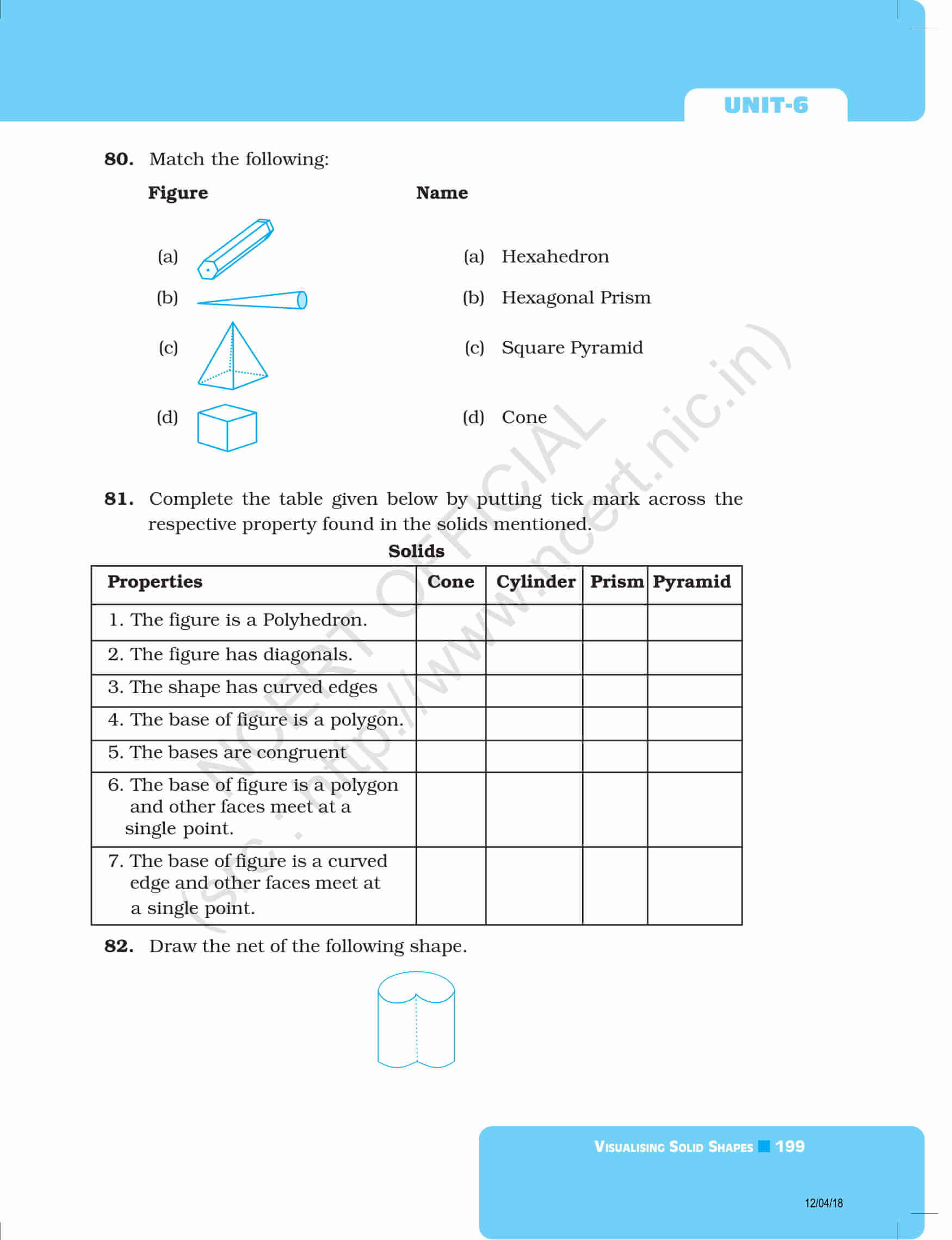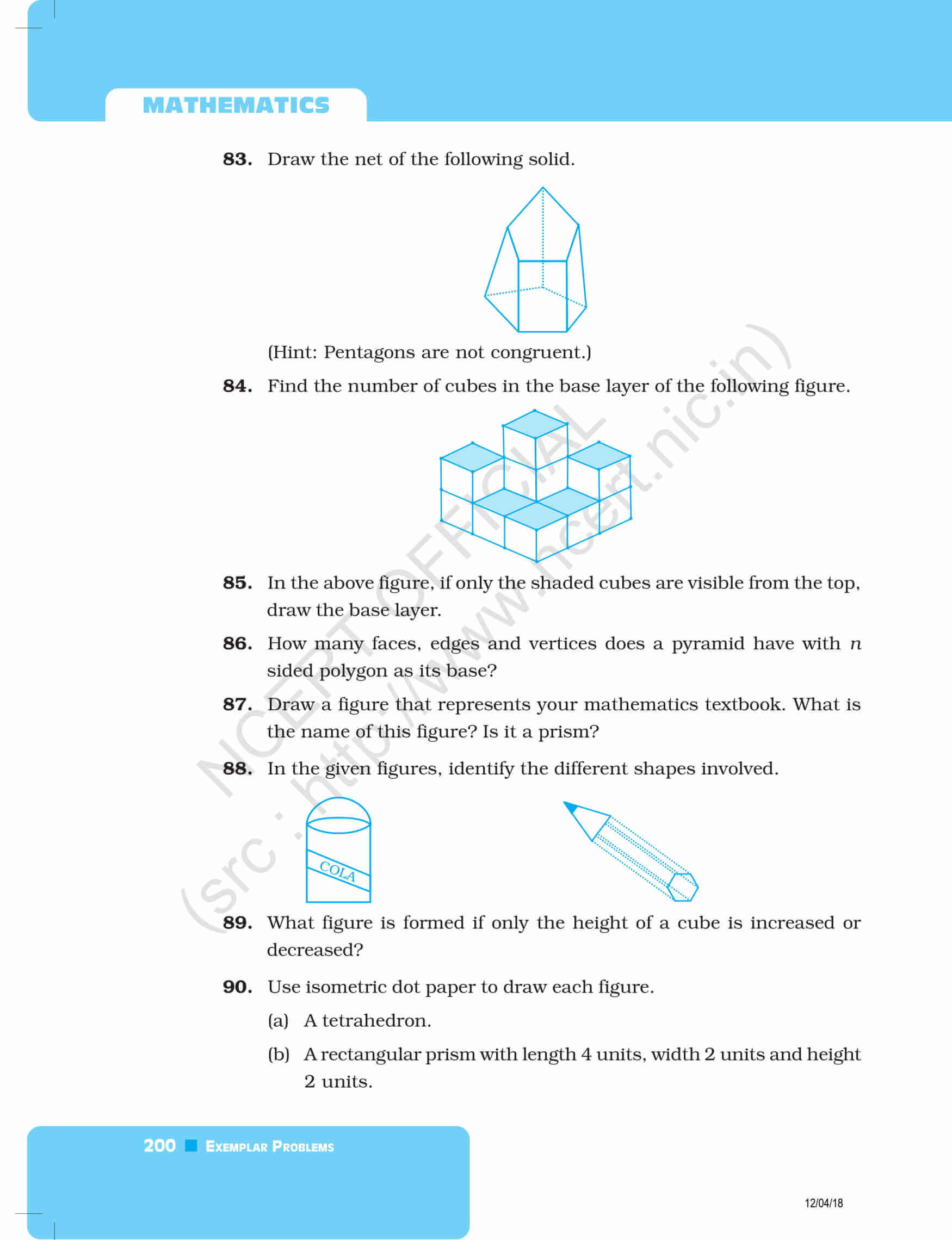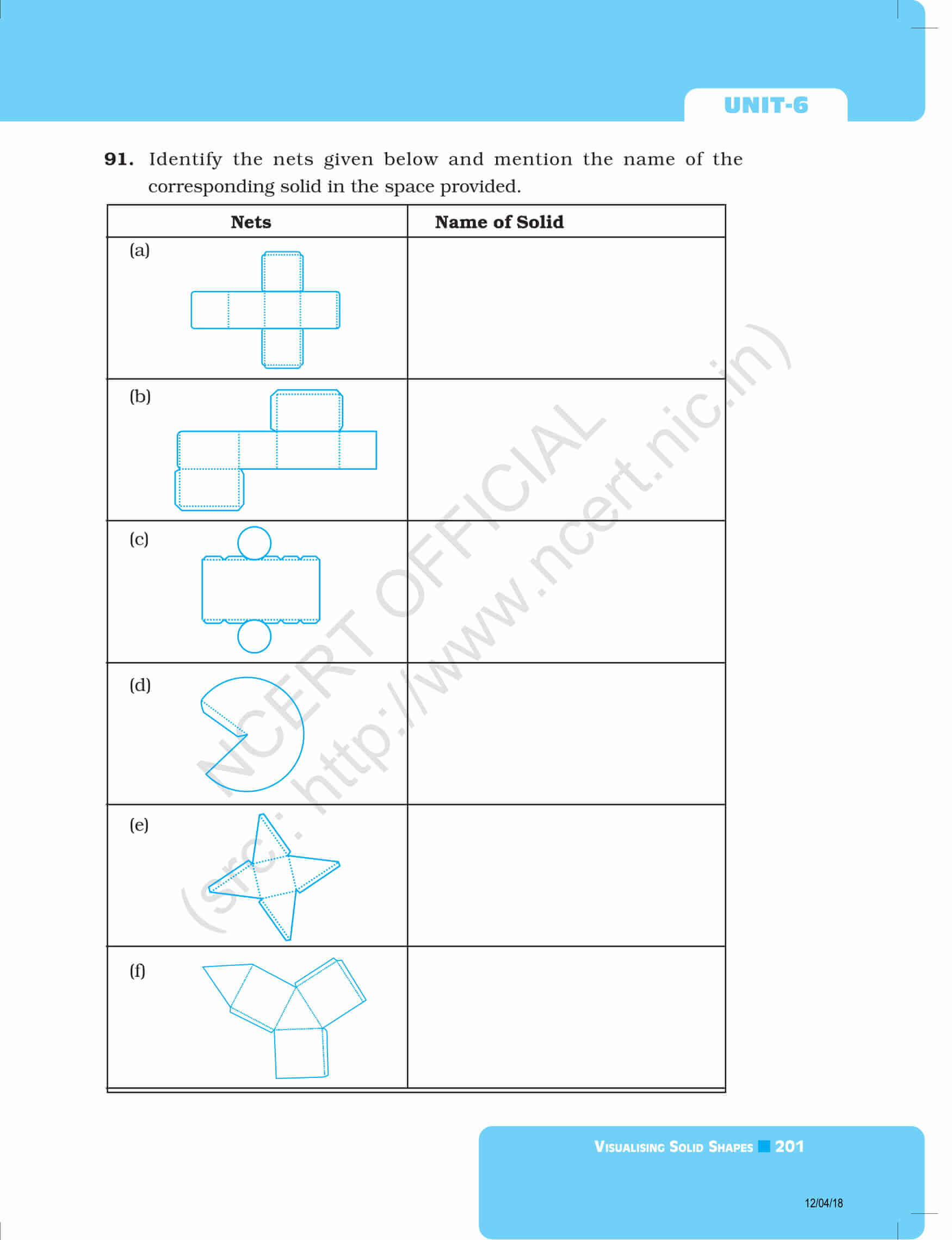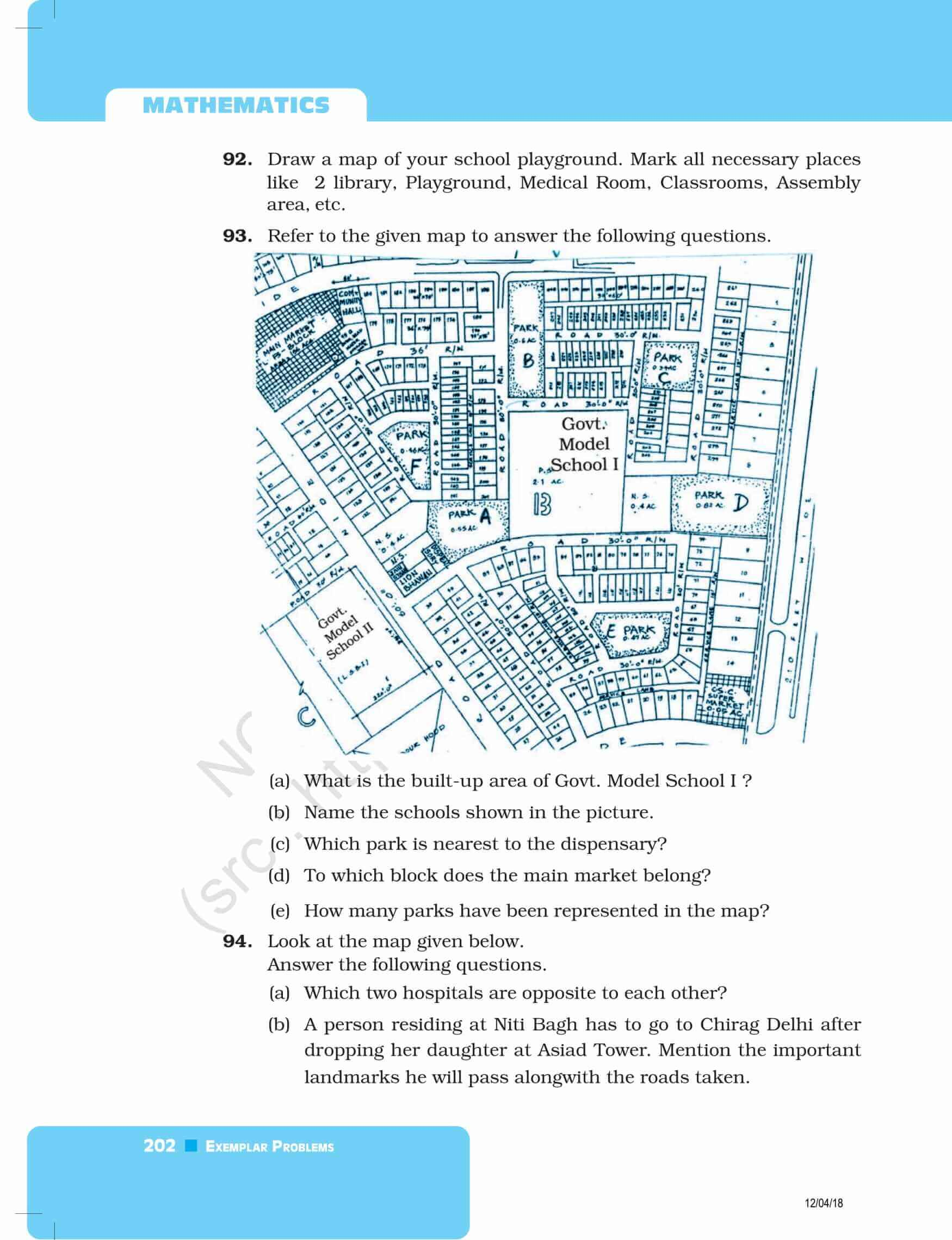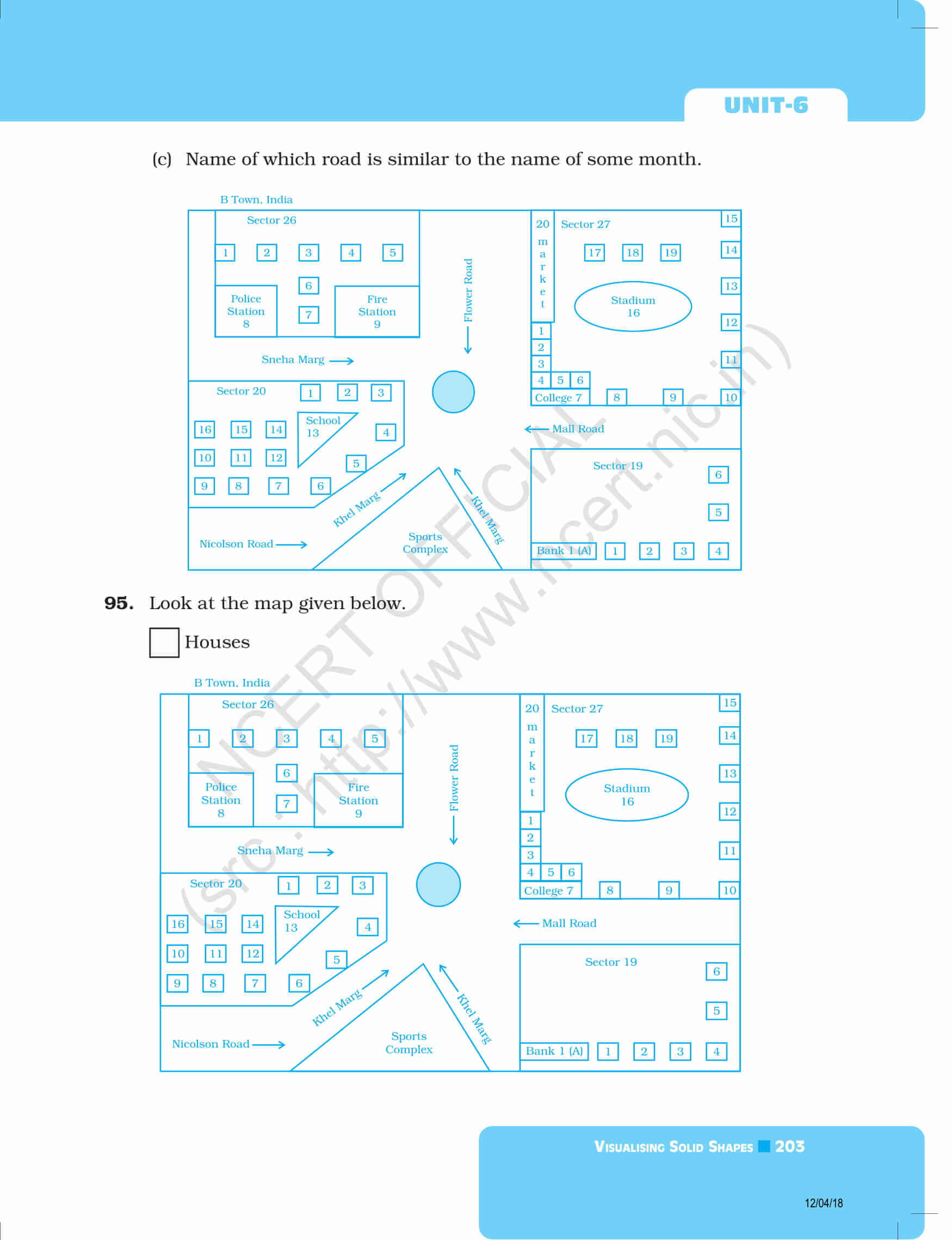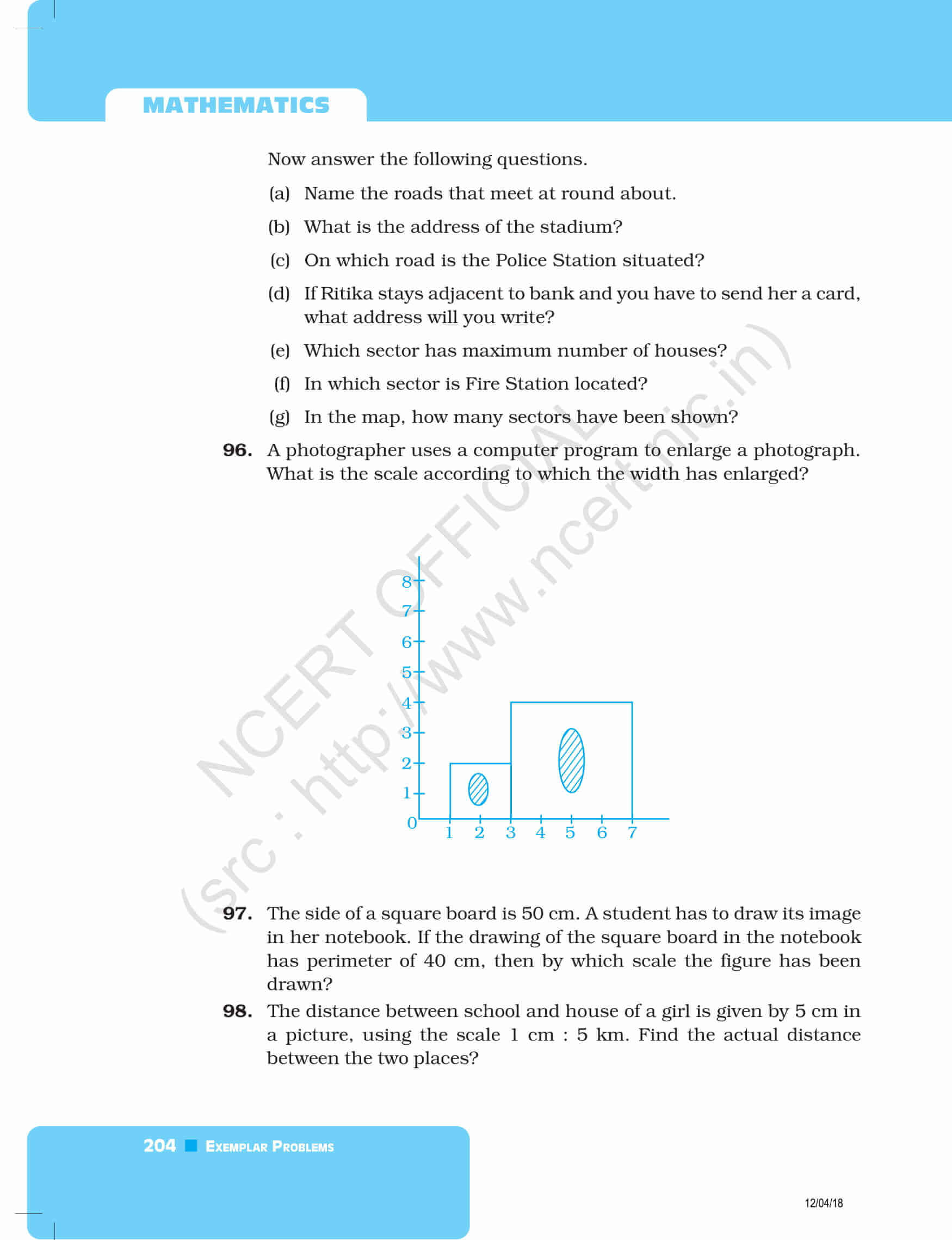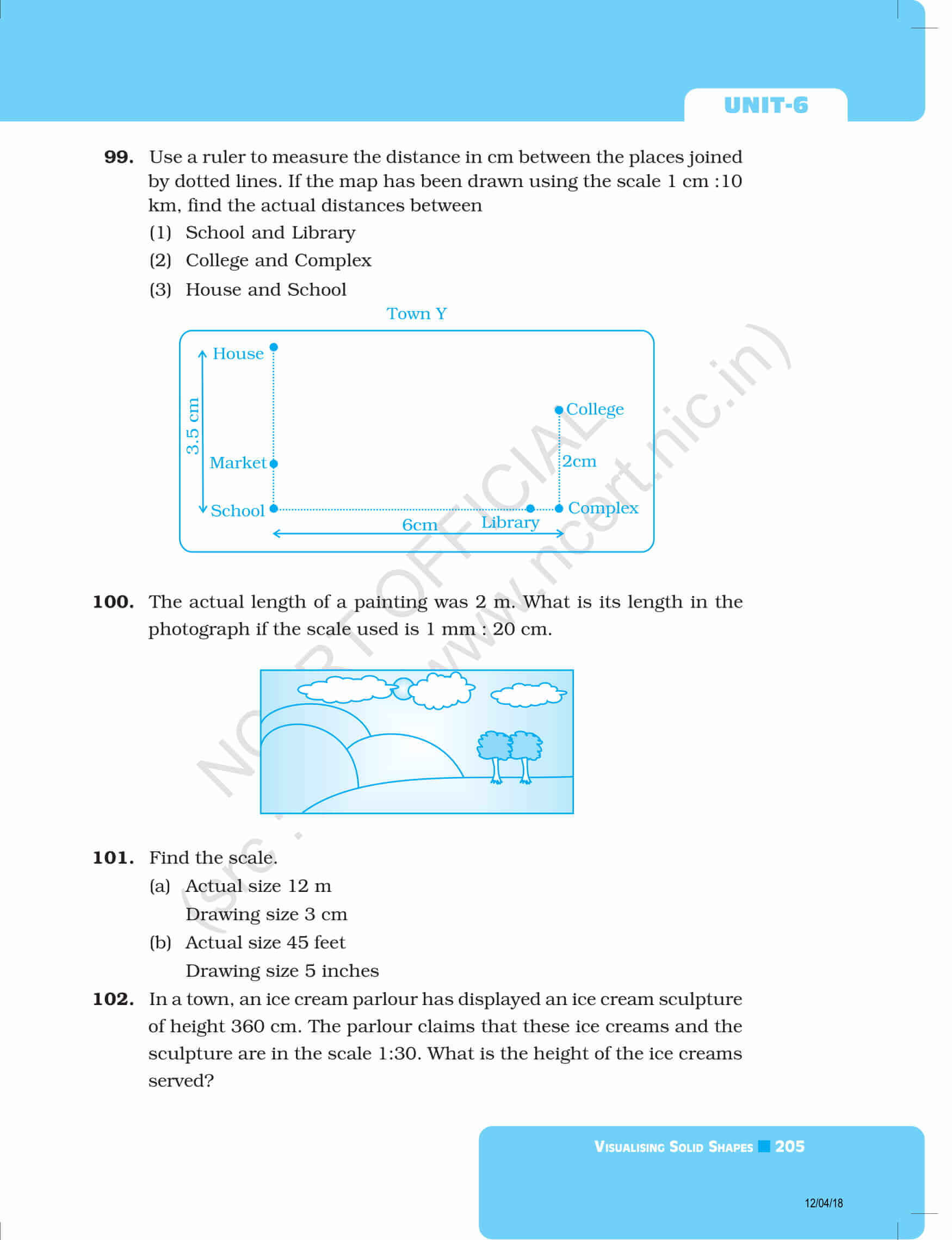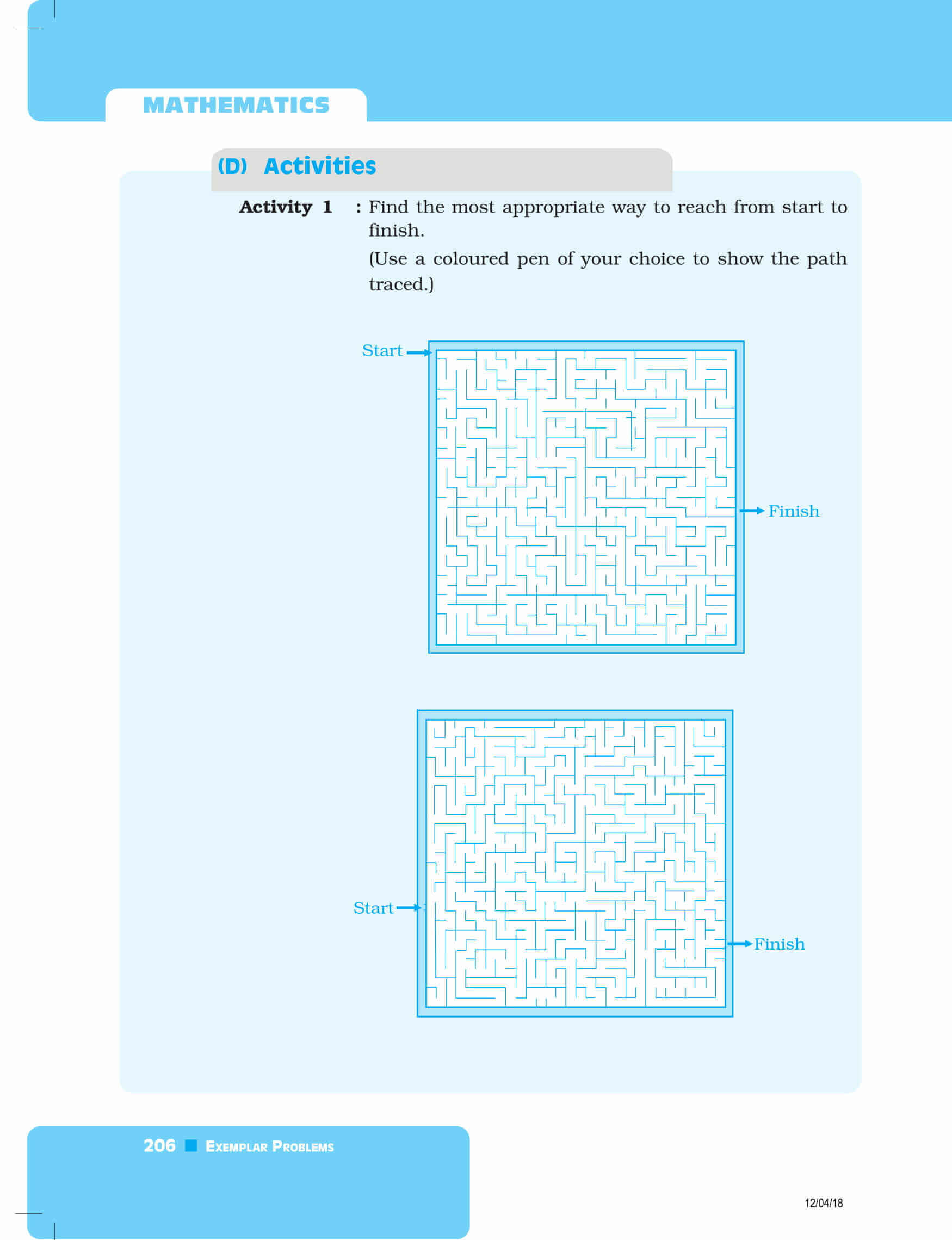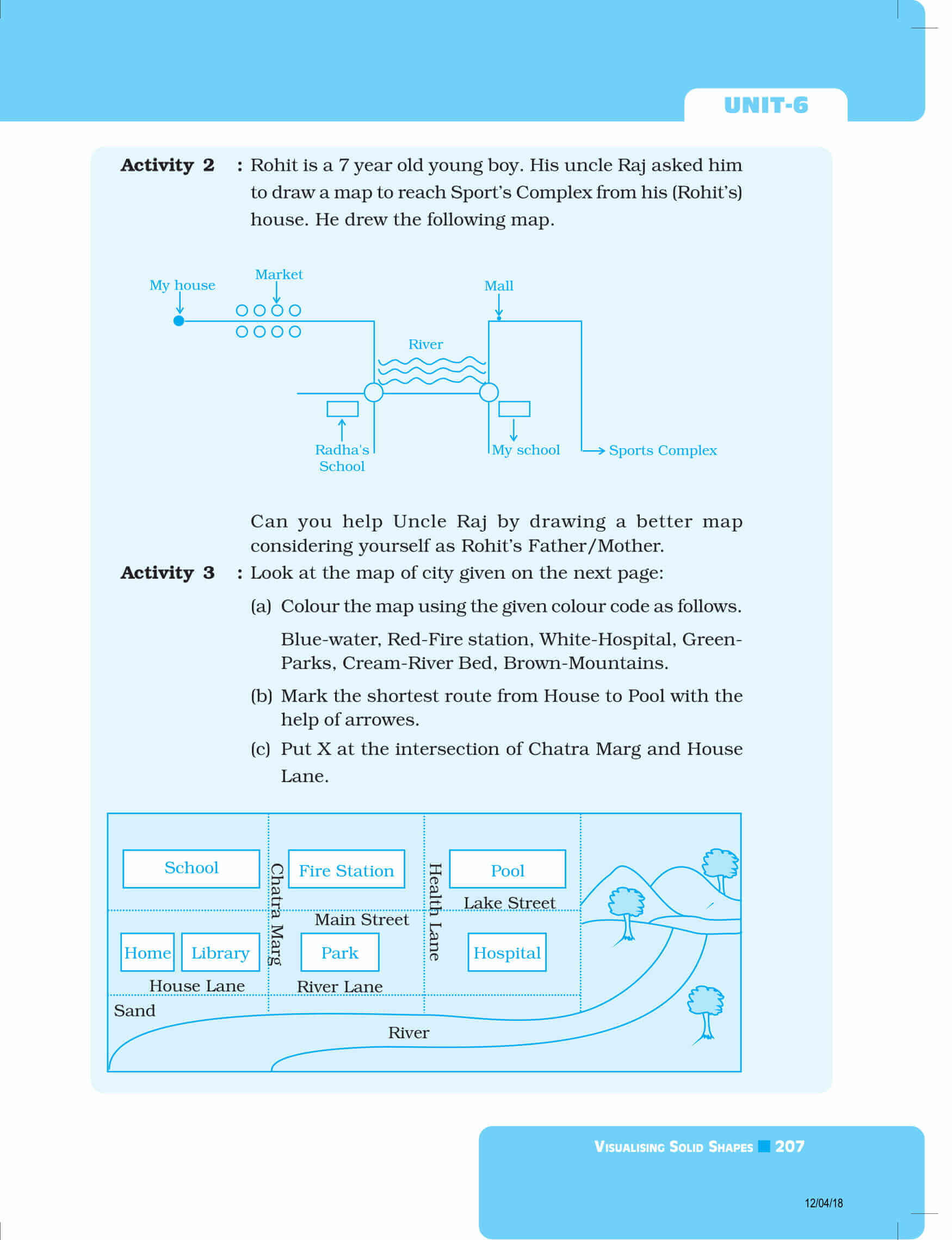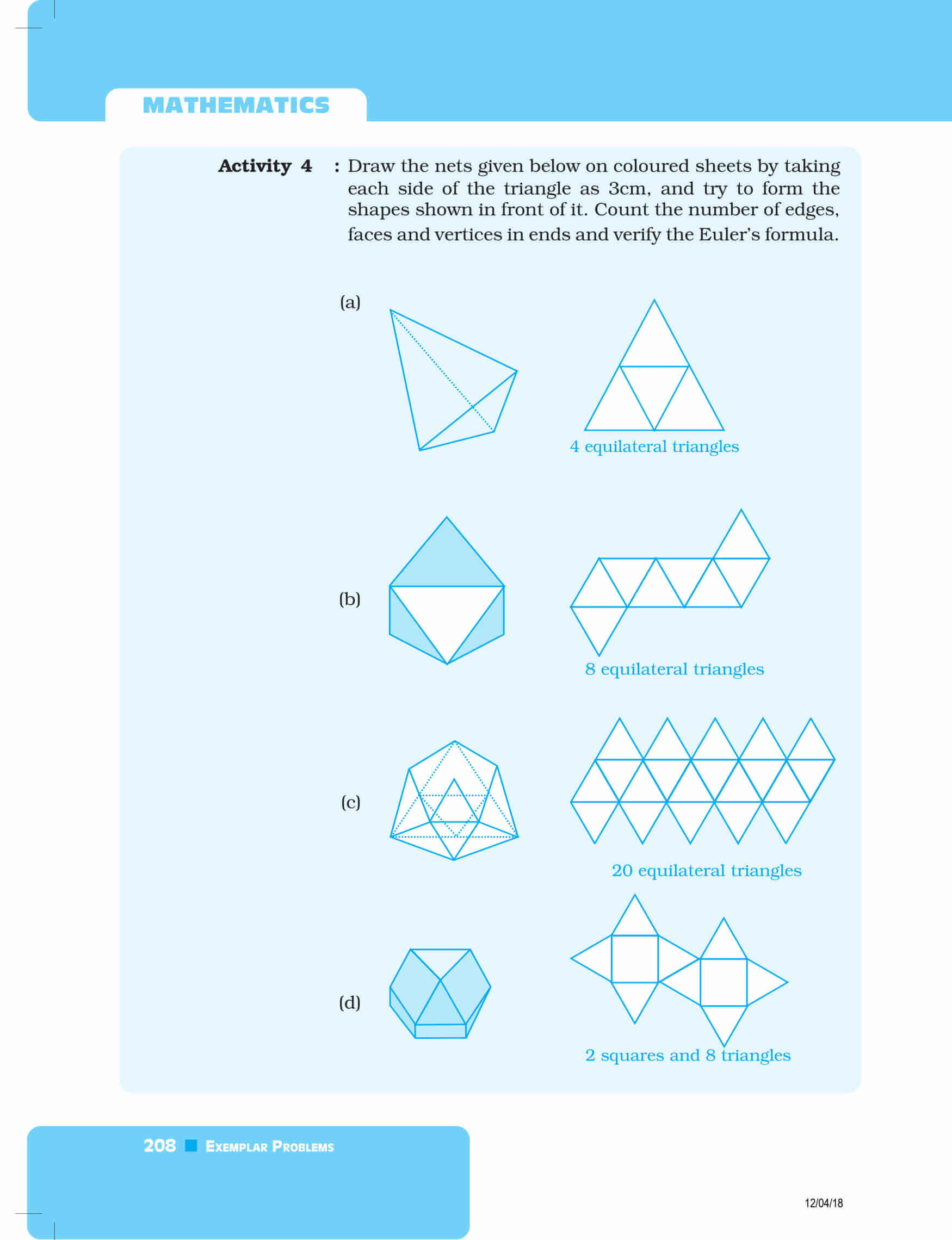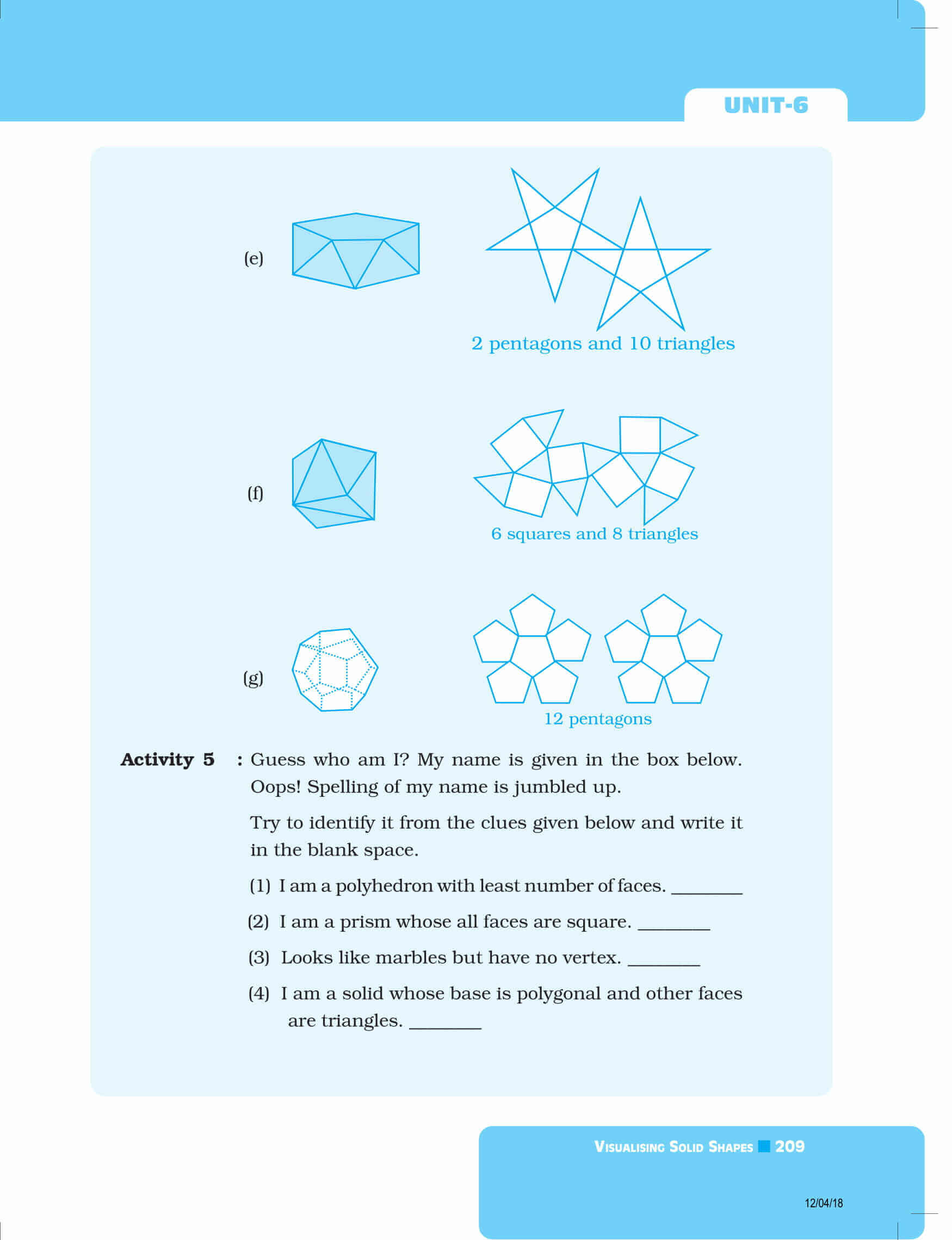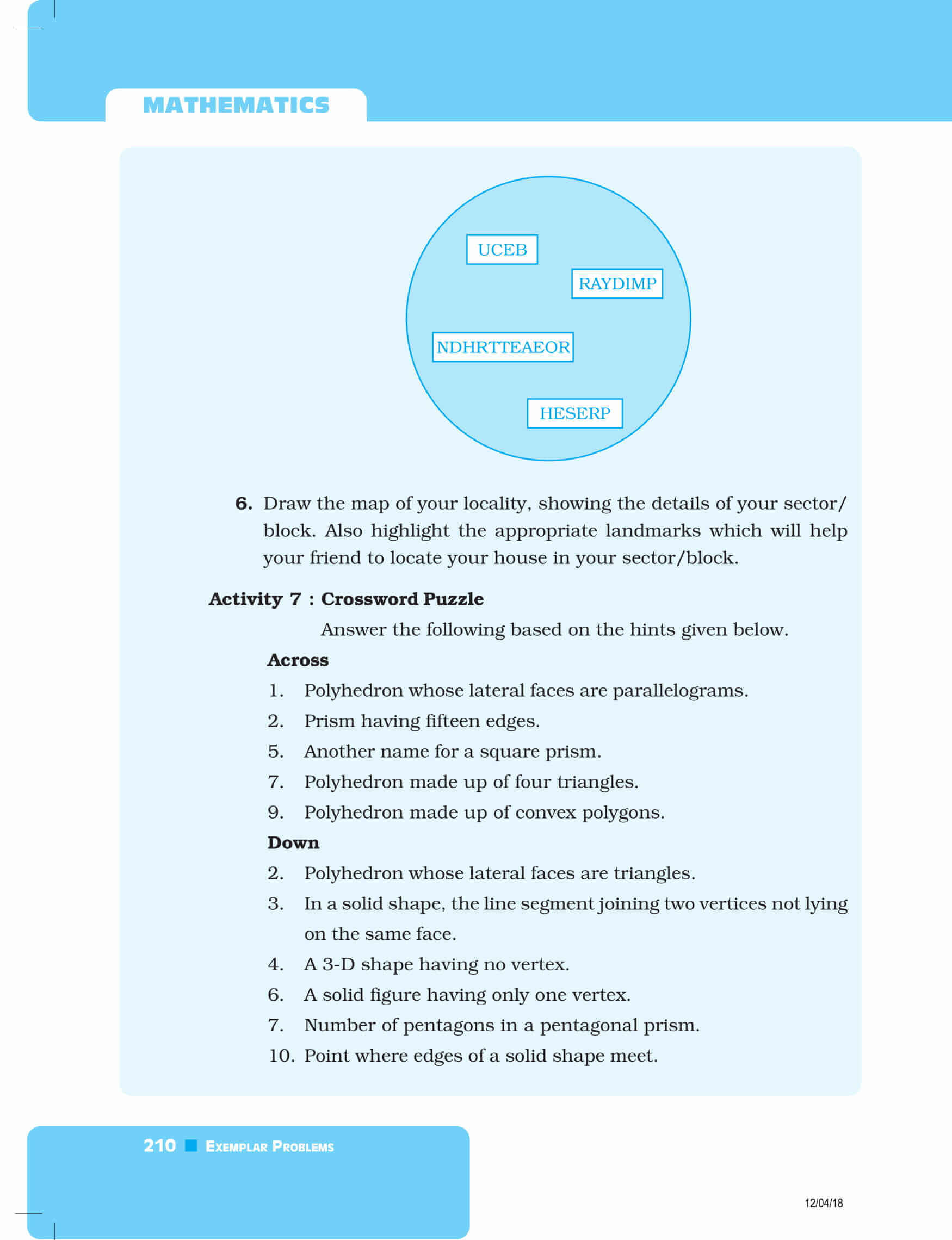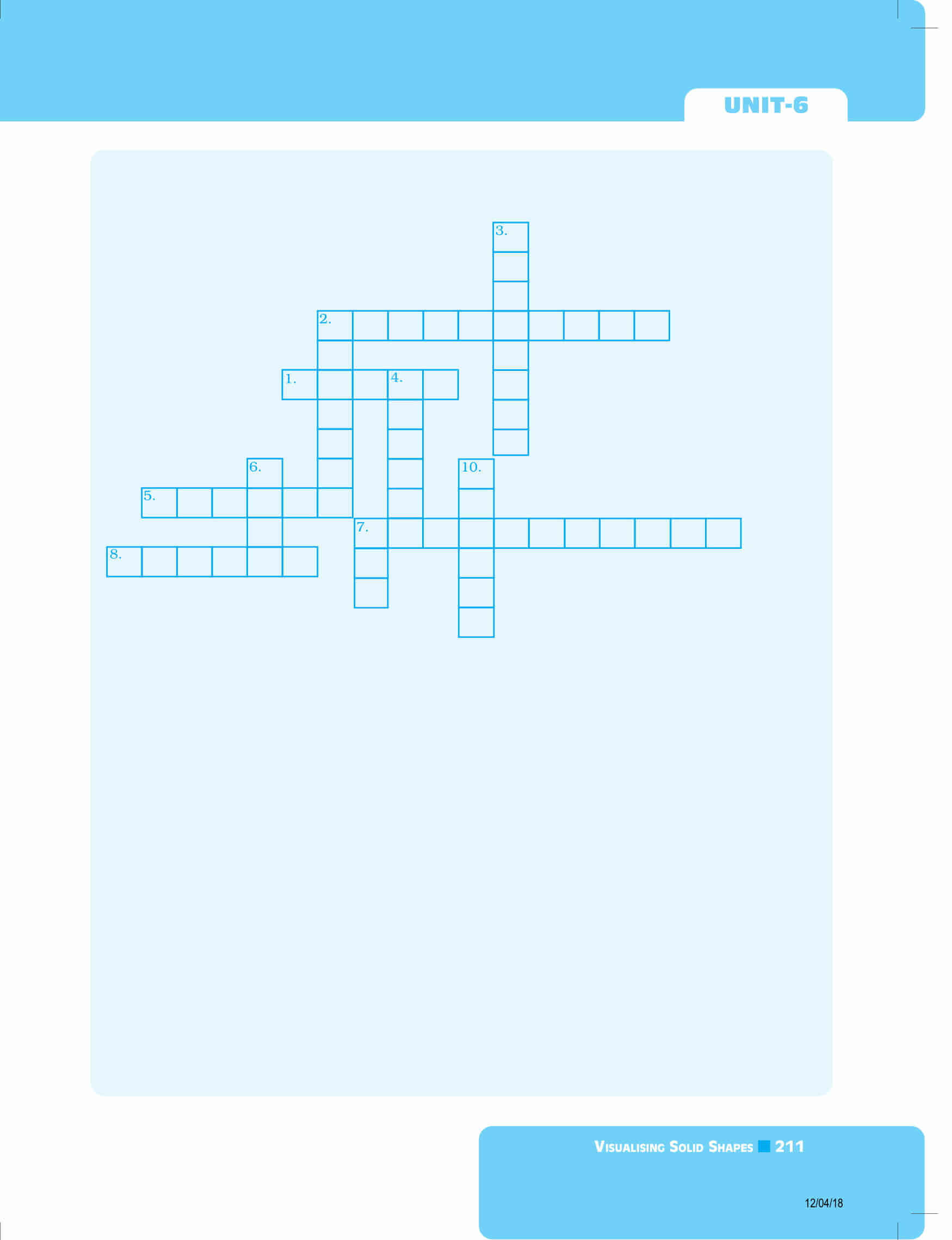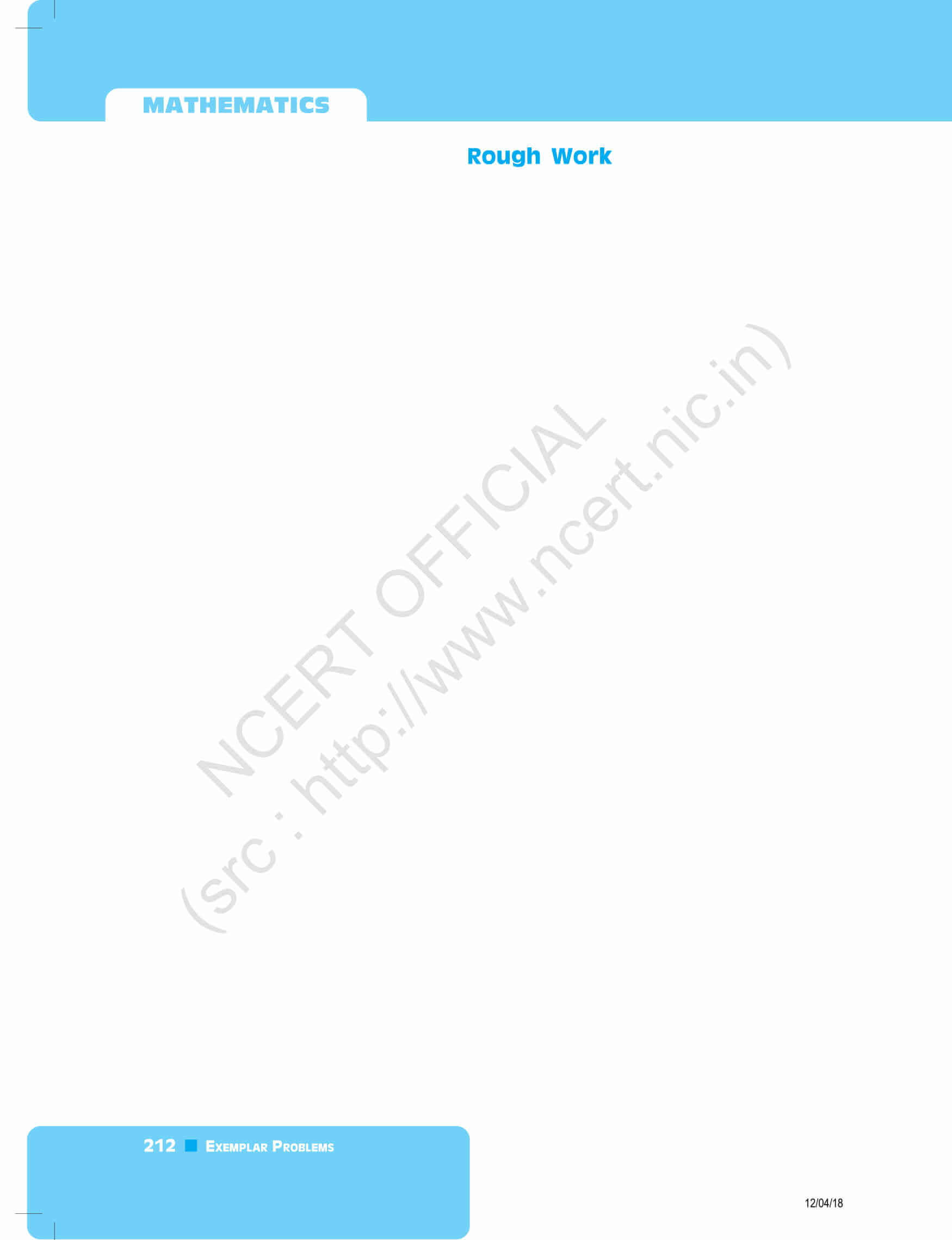To download the exemplars for all chapters of 8th class maths, click here. Download BYJU’S-The Learning App and get personalized videos, explaining the shapes of solids or 2D and 3D figures with the help of pictures and related topics and experience a new way of learning to understand the theories easily.

Also Check